A previous article describes the metalog distribution (Keelin, 2016). The metalog distribution is a flexible family of distributions that can model a wide range of shapes for data distributions. The metalog system can model bounded, semibounded, and unbounded continuous distributions. This article shows how to use the metalog distribution in SAS by downloading a library of SAS IML functions from a GitHub site.

The article contains the following sections:

• An overview of the metalog library of SAS IML functions
• How to download the functions from GitHub and include the functions in a program
• How to use a metalog model to fit an unbounded model to data
• A discussion of infeasible models

For a complete discussion of the metalog functions in SAS, see "A SAS IML Library for the Metalog Distribution" (Wicklin, 2023).

### The metalog library of functions in SAS

The metalog library is a collection of SAS IML modules. The modules are object-oriented, which means that you must first construct a "metalog object," usually from raw data. The object contains information about the model. All subsequent operations are performed by passing the metalog object as an argument to functions. You can classify the functions into four categories:

• Constructors create a metalog object from data. By default, the constructors fit a five-term unbounded metalog model.
• Query functions enable you to query properties of the model.
• Computational functions enable you to compute the PDF and quantiles of the distribution and to generate random variates.
• Plotting routines create graphs of the CDF and PDF of the metalog distribution. The plotting routines are available when you use PROC IML.

Before you create a metalog object, you must choose how to model the data. There are four types of models: unbounded, semibounded with a lower bound, semibounded with an upper bound, or bounded. Then, you must decide the number of terms (k) to use in the metalog model. A small number of terms (3 ≤ k ≤ 6) results in a smooth model. A larger number of terms (k > 6) can fit multimodal data distributions, but be careful not to overfit the data by choosing k too large.

The most common way to create a metalog object is to fit a model to the empirical quantiles of the data, which estimates the k parameters in the model. For an unbounded metalog model, the quantile function is
$Q(p) = a_1 + a_2 L(p) + a_3 c(p) L(p) + a_4 c(p) + a_5 c(p)^2 + a_6 c(p)^2 L(p) + a_7 c(p)^3 + \ldots$
where L(p) = Logit(p) is the quantile function of the logistic distribution and c(p) = p – 1/2 is a linear function. You use ordinary least squares regression to fit the parameters ($a_1, a_2, \ldots, a_k$) in the model.

The semibounded and bounded versions are metalog models of log-transformed variables.

• If X > L, fit a metalog model to Z = log(X – L)
• If L < X < U, fit a metalog model to Z = log((X – L) / (U – X))

The process of downloading SAS code from a GitHub site is explained in the article, "How to use Git to share SAS programs." The process is also explained in Appendix A in the metalog documentation. The following SAS program downloads the metalog functions to the WORK libref. You could use a permanent libref, if you prefer:

/* download metalog package from GitHub */ options dlcreatedir; %let repoPath = %sysfunc(getoption(WORK))/sas-iml-packages; /* clone repo to WORK, or use permanent libref */   /* clone repository; if repository exists, skip download */ data _null_; if fileexist("&repoPath.") then put 'Repository already exists; skipping the clone operation'; else do; put "Cloning repository 'sas-iml-packages'"; rc = gitfn_clone("https://github.com/sassoftware/sas-iml-packages/", "&repoPath." ); end; run;   /* Use %INCLUDE to read source code and STORE functions to current storage library */ proc iml; %include "&repoPath./Metalog/ML_proc.sas"; /* each file ends with a STORE statement */ %include "&repoPath./Metalog/ML_define.sas"; quit;

Each file ends with a STORE statement, so running the above program results in storing the metalog functions in the current storage library. The first file (ML_PROC.sas) should be included only when you are running the program in PROC IML. It contains the plotting routines. The second file (ML_define.sas) should be included in both PROC IML and the iml action.

### Fit an unbounded metalog model to data

The Sashelp.heart data set contains data on patients in a heart study. The data set contains a variable (Systolic) for the systolic blood pressure of 5,209 patients. The following call to PROC SGPLOT creates a histogram of the data and overlays a kernel density estimate.

title 'Systolic Blood Pressure'; proc sgplot data=sashelp.heart; histogram Systolic; density Systolic / type=kernel; keylegend /location=inside; run;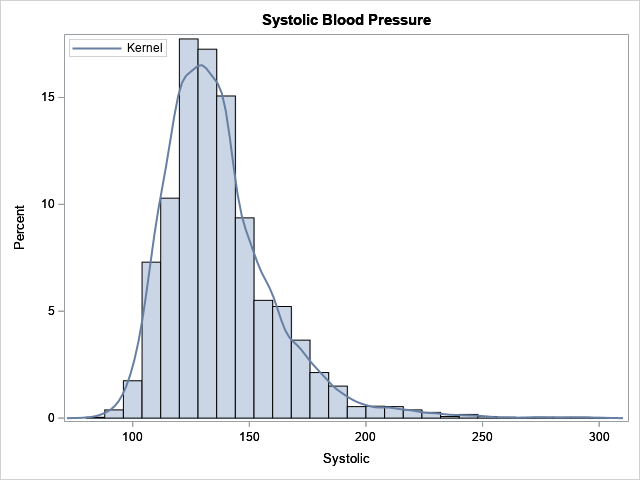The kernel density estimate (KDE) is a nonparametric model. The histogram and the KDE indicate that the data are not normally distributed, and it is not clear whether the data can be modeled by using one of the common "named" probability distributions such as gamma or lognormal. In situations such as this, the metalog distribution can be an effective tool for modeling and simulation. The following SAS IML statements load the metalog library of functions, then fit a five-term unbounded metalog distribution to the data. After you fit the model, it is a good idea to run the ML_Summary subroutine, which displays a summary of the model and displays the parameter estimates.

proc iml; load module=_all_; /* load the metalog library */ use sashelp.heart; read all var "Systolic"; close;   MLObj = ML_CreateFromData(Systolic, 5); /* 5-term unbounded model */ run ML_Summary(MLObj);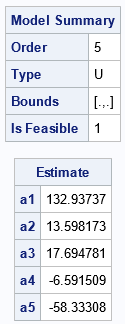The ML_Summary subroutine displays two tables. The first table tells you that the model is a 5-term unbounded model, and it is feasible. Not every model is feasible, which is why it is important to examine the summary of the model. (Feasibility is discussed in a subsequent section.) The second table provides the parameter estimates for the model.

The MLObj variable is an object that encapsulates relevant information about the metalog model. After you create a metalog object, you can pass it to other functions to query, compute, or plot the model. For example, in PROC IML, you can use the ML_PlotECDF subroutine to show the agreement between the cumulative distribution of the model and the empirical cumulative distribution of the data, as follows:

title '5-Term Metalog Model for Systolic Blood Pressure'; run ML_PlotECDF(MLObj);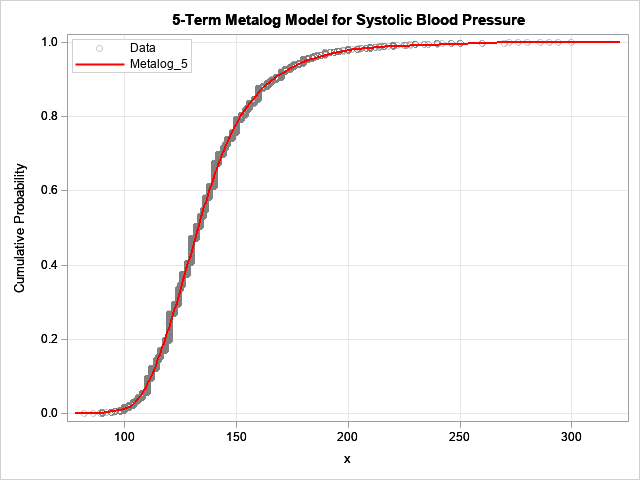The model is fit to 5,209 observations. For this blog post, I drew the CDF of the model in red so that it would stand out. The model seems to fit the data well. If you prefer to view the density function, you can run the statement
run ML_PlotPDF(MLObj);
Again, note that the MLObj variable is passed as the first argument to the function.

One way to assess the goodness of fit is to compare the sample quantiles to the quantiles predicted by the model. You can use the ML_Quantile function to obtain the predicted quantiles, as follows:

/* estimates of quantiles */ Prob = {0.01, 0.05, 0.25, 0.5, 0.75, 0.95, 0.99}; call qntl(SampleEst, Systolic, Prob); /* sample quantiles */ ModelEst = ML_Quantile(MLObj, Prob); /* model quantiles */ print Prob SampleEst ModelEst;The model quantiles agree well with the sample quantiles. Note that the median estimate from the model (the 0.5 quantile) is equal to the a1 parameter estimate. This is always the case.

An advantage of the metalog model is that it has an explicit quantile function. As a consequence, it is easy to simulate from the model by using the inverse CDF method. You can use the ML_Rand function to simulate from the model, as follows:

/* simulate 500 new blood pressure values from the model */ call randseed(1234); title 'Simulated Systolic Values'; BP = ML_Rand(MLObj, 500); call histogram(BP);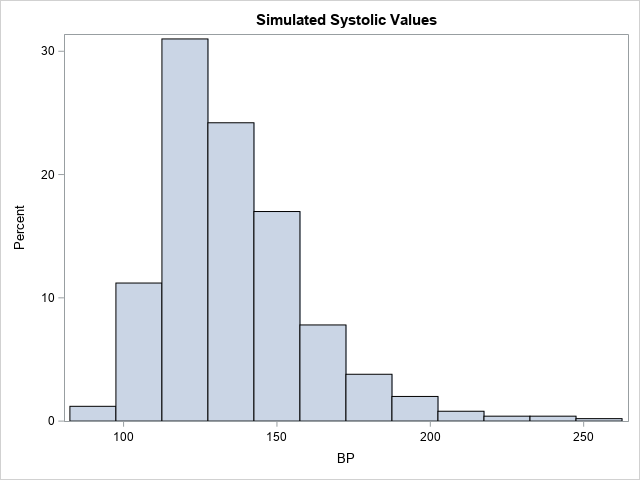Notice that the shape of this histogram is similar to the histogram of the original data. The simulated data seem to have similar properties as the original data.

The metalog documentation provides the syntax and documentation for every public method in the library.

### Infeasible metalog functions

As discussed in a previous article, not every metalog model is a valid distribution. An infeasible model is one for which the quantile function is not monotone increasing. This can happen if you have a small data set that has extreme outliers or gaps, and you attempt to fit a metalog model that has many parameters. The model will overfit the data, which results in an infeasible model.

I was unable to create an infeasible metalog model for the blood-pressure data, which has thousands of observations and no extreme observations. However, if I use only the first 20 observations, I can demonstrate an infeasible model by fitting a six-term metalog model. The following SAS IML statements create a new data vector that contains only 20 observations. A six-term metalog model is fit to those data.

/* What if we use a much smaller data set? Set N = 20. */ S = Systolic[1:20]; /* N = 20 */ MLObj = ML_CreateFromData(S, 6); /* 6-term model */ run ML_Summary(MLObj);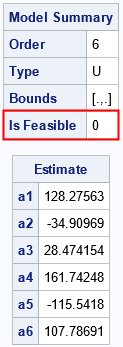As shown by the output of the ML_Summary call, the six-term metalog model is not feasible. The following graph shows the quantile function of the model overlaid on the empirical quantiles. Notice that the quantile function of the model is not monotone increasing, which explains why the model is infeasible. If you encounter this situation in practice, Keelin (2016) suggests reducing the number of terms in the model.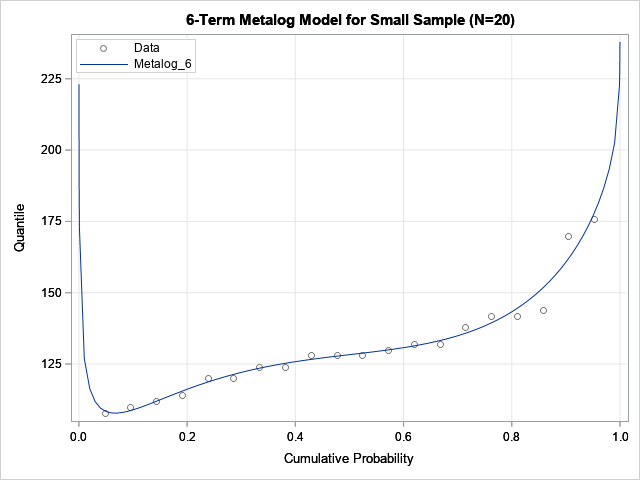### Summary

This article describes how to use the metalog distribution in SAS. The metalog distribution is a flexible family that can model a wide range of distributional shapes. You can download the Metalog package from the SAS Software GitHub site. The package contains documentation and a collection of SAS IML modules. You can call the modules in a SAS IML program to fit a metalog model. After fitting the model, you can compute properties of the model and simulate data from the model. More information and examples are available in the documentation of the Metalog package.

The post Use the metalog distribution in SAS appeared first on The DO Loop.

A previous article shows that you can use the Intercept parameter to control the ratio of events to nonevents in a simulation of data from a logistic regression model. If you decrease the intercept parameter, the probability of the event decreases; if you increase the intercept parameter, the probability of the event increases.

The probability of Y=1 also depends on the slope parameters (coefficients of the regressor effects) and on the distribution of the explanatory variables. This article shows how to visualize the probability of an event as a function of the regression parameters. I show the visualization for two one-variable logistic models. For the first model, the distribution of the explanatory variable is standard normal. In the second model, the distribution is exponential.

### Visualize the probability as the Intercept varies

In a previous article, I simulated data from a one-variable binary logistic model. I simulated the explanatory variable, X, from a standard normal distribution. The linear predictor is given by η = β0 + β1 X. The probability of the event Y=1 is given by μ = logistic(η) = 1 / (1 + exp(-η)).

In the previous article, I looked at various combinations of Intercept (β0) and Slope (β1) parameters. Now, let's look systematically at how the probability of Y=1 depends on the Intercept for a fixed value of Slope > 0. Most of the following program is repeated and explained in my previous post. For your convenience, it is repeated here. First, the program simulates data (N=1000) for a variable, X, from a standard normal distribution. Then it simulates a binary variable for a series of binary logistic models as the intercept parameter varies on the interval [-3, 3]. (To get better estimates of the probability of Y=1, the program simulates 100 data sets for each value of Intercept.) Lastly, the variable calls PROC FREQ to compute the proportion of simulated events (Y=1), and it plots the proportion versus the Intercept value.

/* simulate X ~ N(0,1) */ data Explanatory; call streaminit(12345); do i = 1 to 1000; x = rand("Normal", 0, 1); /* ~ N(0,1) */ output; end; drop i; run;   /* as Intercept changes, how does P(Y=1) change when Slope=2? */ %let Slope = 2; data SimLogistic; call streaminit(54321); set Explanatory; do Intercept = -3 to 3 by 0.2; do nSim = 1 to 100; /* Optional: param estimate is better for large samples */ eta = Intercept + &Slope*x; /* eta = linear predictor */ mu = logistic(eta); /* mu = Prob(Y=1) */ Y = rand("Bernoulli", mu); /* simulate binary response */ output; end; end; run;   proc sort data=SimLogistic; by Intercept; run; ods select none; proc freq data=SimLogistic; by Intercept; tables Y / nocum; ods output OneWayFreqs=FreqOut; run; ods select all;   title "Percent of Y=1 in Logistic Model"; title2 "Slope=&Slope"; footnote J=L "X ~ N(0,1)"; proc sgplot data=FreqOut(where=(Y=1)); series x=Intercept y=percent; xaxis grid; yaxis grid label="Percent of Y=1"; run;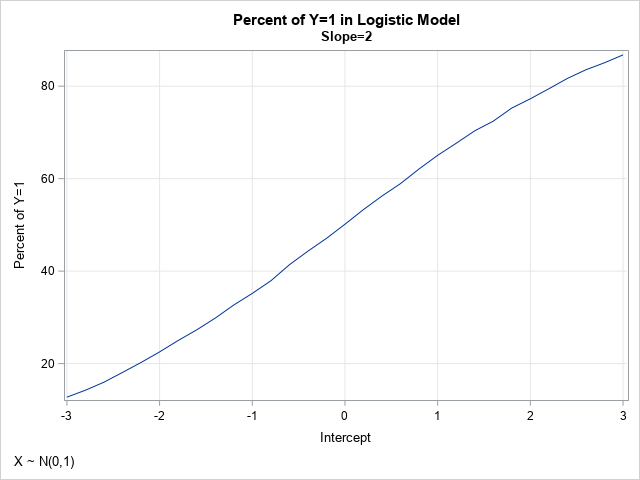In the simulation, the slope parameter is set to Slope=2, and the Intercept parameter varies systematically between -3 and 3. The graph visualizes the probability (as a percentage) that Y=1, given various values for the Intercept parameter. This graph depends on the value of the slope parameter and on the distribution of the data. It also has random variation, due to the call to generate Y as a random Bernoulli variate. For these data, the graph shows the estimated probability of the event as a function of the Intercept parameter. When Intercept = –2, Pr(Y=1) = 22%; when Intercept = 0, Pr(Y=1) = 50%; and when Intercept = 2, Pr(Y=1) = 77%. The statement that Pr(Y=1) = 50% when Intercept=0 should be approximately true when the data is from a symmetric distribution with zero mean.

### Vary the slope and intercept together

In the previous section, the slope parameter is fixed at Slope=2. What if we allow the slope to vary over a range of values? We can restrict our attention to positive slopes because logistic(η) = 1 – logistic(-η). The following program varies the Intercept parameter in the range [-3,3] and the Slope parameter in the range [0, 4].

/* now do two-parameter simulation study where slope and intercept are varied */ data SimLogistic2; call streaminit(54321); set Explanatory; do Slope = 0 to 4 by 0.2; do Intercept = -3 to 3 by 0.2; do nSim = 1 to 50; /* optional: the parameter estimate are better for larger samples */ eta = Intercept + Slope*x; /* eta = linear predictor */ mu = logistic(eta); /* transform by inverse logit */ Y = rand("Bernoulli", mu); /* simulate binary response */ output; end; end; end; run;   /* Monte Carlo estimate of Pr(Y=1) for each (Int,Slope) pair */ proc sort data=SimLogistic2; by Intercept Slope; run; ods select none; proc freq data=SimLogistic2; by Intercept Slope; tables Y / nocum; ods output OneWayFreqs=FreqOut2; run; ods select all;

The output data set (FreqOut2) contains Monte Carlo estimates of the probability that Y=1 for each pair of (Intercept, Slope) parameters, given the distribution of the explanatory variable. You can use a contour plot to visualize the probability of the event for each combination of slope and intercept:

/* Create template for a contour plot https://blogs.sas.com/content/iml/2012/07/02/create-a-contour-plot-in-sas.html */ proc template; define statgraph ContourPlotParm; dynamic _X _Y _Z _TITLE _FOOTNOTE; begingraph; entrytitle _TITLE; entryfootnote halign=left _FOOTNOTE; layout overlay; contourplotparm x=_X y=_Y z=_Z / contourtype=fill nhint=12 colormodel=twocolorramp name="Contour"; continuouslegend "Contour" / title=_Z; endlayout; endgraph; end; run; /* render the Monte Carlo estimates as a contour plot */ proc sgrender data=Freqout2 template=ContourPlotParm; where Y=1; dynamic _TITLE="Percent of Y=1 in a One-Variable Logistic Model" _FOOTNOTE="X ~ N(0, 1)" _X="Intercept" _Y="Slope" _Z="Percent"; run;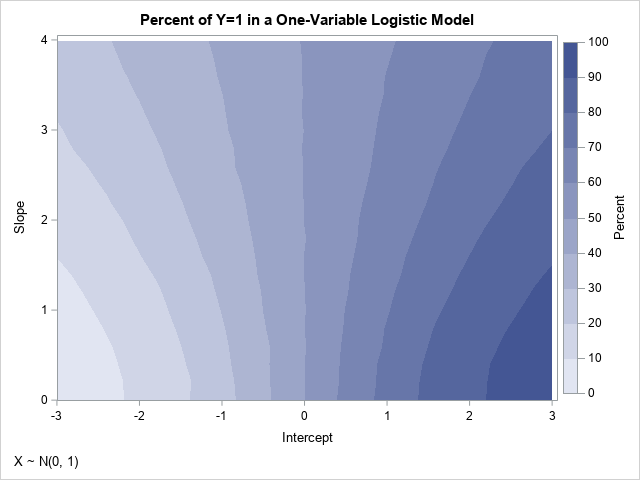As mentioned earlier, because X is approximately symmetric, the contours of the graph have reflective symmetry. Notice that the probability that Y=1 is 50% whenever Intercept=0 for these data. Furthermore, if the points (β0, β1) are on the contour for Pr(Y=1)=α, then the contour for Pr(Y=1)=1-α contains points close to (-β0, β1). The previous line plot is equivalent to slicing the contour plot along the horizontal line Slope=2.

You can use a graph like this to simulate data that have a specified probability of Y=1. For example, if you want approximately 70% of the cases to be Y=1, you can choose any pair of (Intercept, Slope) values along that contour, such as (0.8, 0), (1, 1), (2, 3.4), and (2.4, 4). If you want to see all parameter values for which Pr(Y=1) is close to a desired value, you can use the WHERE statement in PROC PRINT. For example, the following call to PROC PRINT displays all parameter values for which the Pr(Y=1) is approximately 0.7 or 70%:

%let Target = 70; proc print data=FreqOut2; where Y=1 and %sysevalf(&Target-1) <= Percent <= %sysevalf(&Target+1); var Intercept Slope Y Percent; run;

### Probabilities for nonsymmetric data

The symmetries in the previous graphs are a consequence of the symmetry in the data for the explanatory variable. To demonstrate how the graphs change for a nonsymmetric data distribution, you can run the same simulation study, but use data that are exponentially distributed. To eliminate possible effects due to a different mean and variance in the data, the following program standardizes the explanatory variable so that it has zero mean and unit variance.

data Expo; call streaminit(12345); do i = 1 to 1000; x = rand("Expon", 1.5); /* ~ Exp(1.5) */ output; end; drop i; run;   proc stdize data=Expo method=Std out=Explanatory; var x; run;

If you rerun the simulation study by using the new distribution of the explanatory variable, you obtain the following contour plot of the probability as a function of the Intercept and Slope parameters: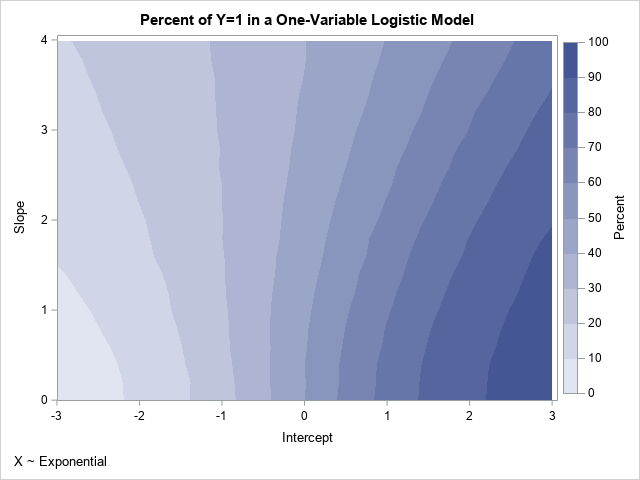If you compare this new contour plot to the previous one, you will see that they are very similar for small values of the slope parameter. However, they are different for larger values such as Slope=2. The contours in the new plot do not show reflective symmetry about the vertical line Intercept=0. Pairs of parameters for which Pr(Y=1) is approximately 0.7 include (0.8, 0) and (1, 1), which are the same as for the previous plot, and (2.2, 3.4), and (2.6, 4), which are different from the previous example.

### Summary

This article presents a Monte Carlo simulation study in SAS to compute and visualize how the Intercept and Slope parameters of a one-variable logistic model affect the probability of the event. A previous article notes that decreasing the Intercept decreases the probability. This article shows that the probability depends on both the Intercept and Slope parameters. Furthermore, the probability depends on the distribution of the explanatory variables. You can use the results of the simulation to control the proportion of events to nonevents in the simulated data.

The ideas in this article generalize to logistic regression models that contain multiple explanatory variables. For multivariate models, the effect of the Intercept parameter is similar. However, the effect of the slope parameters is more complicated, especially when the variables are correlated with each other.

This article shows that you can use the intercept parameter to control the probability of the event in a simulation study that involves a binary logistic regression model. For simplicity, I will simulate data from a logistic regression model that involves only one explanatory variable, but the main idea applies to multivariate regression models. I also show mathematically why the intercept parameter controls the probability of the event.

### The problem: Unbalance events when simulating regression data

Usually, simulation studies are based on real data. From a set of data, you run a statistical model that estimates parameters. Assuming the model fits the data, you can simulate new data by using the estimates as if they were the true parameters for the model. When you start with real data, you obtain simulated data that often have similar statistical properties.

However, sometimes you might want to simulate data for an example, and you don't have any real data to model. This is often the case on online discussion forums such as the SAS Support Community. Often, a user posts a question but cannot post the data because it is proprietary. In that case, I often simulate data to demonstrate how to use SAS to answer the question. The simulation is usually straightforward for univariate data or for data from an ordinary least squares regression model. However, the simulation can become complicated for generalized linear regression models and nonlinear models. Why? Because not every choice of parameters leads to a reasonable data set. For example, if you choose parameters "out of thin air" in an arbitrary manner, you might end up with a model for which the probability of the event (Pr(Y=1)) is close to 1 for all values of the explanatory variables. This model is not very useful.

It turns out that you can sometimes "fix" your model by decreasing the value of the intercept parameter in the model. This reduces the value of Pr(Y=1), which can lead to a more equitable and balanced distribution of the response values.

### Simulate and visualize a one-variable binary logistic regression model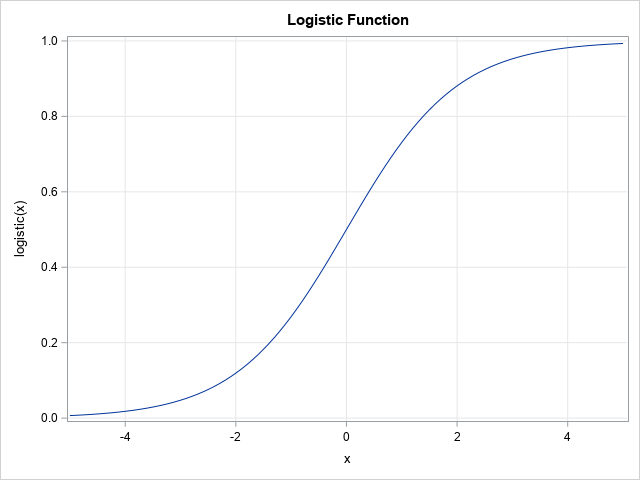Let's construct an example. For a general discussion and a multivariate example, see the article, "Simulating data for a logistic regression model." For this article, let's keep it simple and use a one-variable model. Let X be an independent variable that is distributed as a standardized normal variate: X ~ N(0,1). Then choose an intercept parameter, β0, and a slope parameter, β1, and form the linear predictor η = β0 + β1 X. A binary logistic model uses a logistic transformation to transform the linear predictor to a probability: μ = logistic(η), where logistic(η) = 1 / (1 + exp(-η)). The graph of the S-shaped logistic function is shown to the right. You can then simulate a response as a Bernoulli random variable Y ~ Bern(μ).

In SAS, the following DATA step simulates data from a standard normal variable:

data Explanatory; call streaminit(12345); do i = 1 to 1000; x = rand("Normal", 0, 1); /* ~ N(0,1) */ output; end; drop i; run;

Regardless of the distribution of X, the following DATA step simulates data from a logistic model that has a specified set of values for the regression parameters. I embed the program in a SAS macro so that I can run several models with different values of the Intercept and Slope parameters. The macro also runs PROC FREQ to determine the relative proportions of the binary response Y=0 and Y=1. Lastly, the macro calls PROC SGPLOT to display a graph that shows the logistic regression model and the "observed" responses as a function of the linear predictor values,

/* Simulate data from a one-variable logistic model. X ~ N(0,1) and Y ~ Bern(eta) where eta = logistic(Intercept + Slope*X). Display frequency table for Y and a graph of Y and Prob(Y=1) vs eta */ %macro SimLogistic(Intercept, Slope); title "Logistic Model for Simulated Data"; title2 "Intercept=&Intercept; Slope=&Slope"; data Sim1; call streaminit(54321); set Explanatory; eta = &Intercept + &Slope*x; /* eta = linear predictor */ mu = logistic(eta); /* mu = Prob(Y=1) */ Y = rand("Bernoulli", mu); /* simulate binary response */ run;   proc freq data=Sim1; tables Y / nocum; run; proc sort data=Sim1; by eta; run;   proc sgplot data=Sim1; label Y="Observed" mu="P(Y=1)" eta="Linear Predictor"; scatter x=eta y=Y / transparency=0.8 markerattrs=(symbol=CircleFilled); series x=eta y=mu; xaxis grid values = (-16 to 16) valueshint; yaxis grid label="Probability"; keylegend / location=inside position=E opaque; run; %mend;

### How does the probability of the event depend on the intercept?

Let's run the program. The following call uses an arbitrary choice of values for the regression parameters: Intercept=5 and Slope=2:

/* call the macro with Intercept=5 and Slope=2 */ %SimLogistic(5, 2);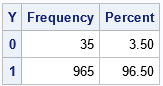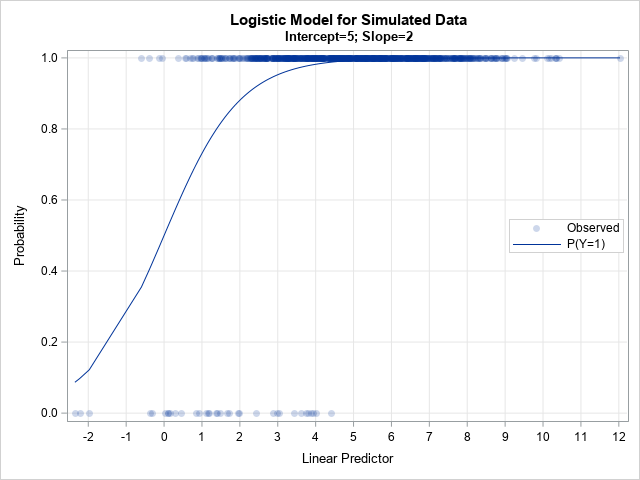The output shows what can happen if you aren't careful when choosing the regression parameters. For this simulation, 96.5% of the response values are Y=1 and only a few are Y=0. This could be a satisfactory model for a rare event, but not for situations in which the ratio of events to nonevents is more balanced, such as 75-25, 60-40, or 50-50 ratios.

The graph shows the problem: the linear predictor (in matrix form, η = X*β) is in the range [-2, 12], and about 95% of the linear prediction are greater than 1.5. Because logistic(1.5) > 0.8, the probability of Y=1 is very high for most observations.

Since you have complete control of the parameters in the simulation, you can adjust the parameters. If you shift η to the left, the corresponding probabilities will decrease. For example, if you subtract 4 from η, then the range of η becomes [-6, 8], which should provide a more balanced distribution of the response category. The following statement implements this choice:

/* call the macro with Intercept=1 and Slope=2 */ %SimLogistic(1, 2);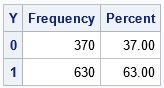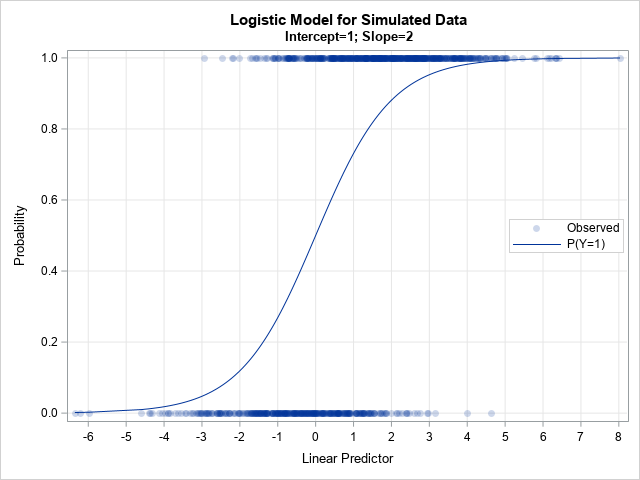The output shows the results of the simulation after decreasing the intercept parameter. When the Intercept=1 (instead of 5), the proportion of simulated responses is more balanced. In this case, 63% of the responses are Y=1 and 37% are Y=0. If you want to further decrease the proportion of Y=1, you can decrease the Intercept parameter even more. For example, if you run %SimLogistic(-1, 2), then only 35.9% of the simulated responses are Y=1.

### The mathematics

I have demonstrated that decreasing η (the linear predictor) also decreases μ (the probability of Y=1). This is really a simple mathematical consequence of the formula for the logistic function. Suppose that η1 < η2. Then -η1 > -η2
⇒    1 + exp(-η1) > 1 + exp(-η2) because EXP is a monotonic increasing function
⇒    1 / (1 + exp(-η1)) < 1 / (1 + exp(-η2)) because the reciprocal function is monotonic decreasing
⇒    μ1 < μ2 by definition.
Therefore, decreasing η results in decreasing μ For some reason, visualizing the simulation makes more of an impact than the math.

### Summary

This article shows that by adjusting the value of the Intercept parameter in a simulation study, you can adjust the ratio of events to nonevents. Decrease the intercept parameter to decrease the number of events; increase the intercept parameter to increase the number of events. I demonstrated this fact for a one-variable model, but the main ideas apply to multivariate models for which the linear predictor (η) is a linear combination of multiple variables.

The probability of Y=1 depends continuously on the regression parameters because the linear predictor and the logistic function are both continuous. In my next article, I show how to visualize the probability of an event as a function of the regression parameters.

I recently gave a presentation about the SAS/IML matrix language in which I emphasized that a matrix language enables you to write complex analyses by using only a few lines of code. In the presentation, I used least squares regression as an example. One participant asked how many additional lines of code would be required for binary logistic regression. I think my answer surprised him. I claimed it would take about a dozen lines of code to obtain parameter estimates for logistic regression. This article shows how to obtain the parameter estimates for a logistic regression model "manually" by using maximum likelihood estimation.

### Example data and logistic regression model

Of course, if you want to fit a logistic regression model in SAS, you should use PROC LOGISTIC or another specialized regression procedure. The LOGISTIC procedure not only gives parameter estimates but also produces related statistics and graphics. However, implementing a logistic regression model from scratch is a valuable exercise because it enables you to understand the underlying statistical and mathematical principles. It also enables you to understand how to generalize the basic model. For example, with minimal work, you can modify the program to support binomial data that represent events and trials.

Let's start by running PROC LOGISTIC on data from the PROC LOGISTIC documentation. The response variable, REMISS, indicates whether there was cancer remission in each of 27 cancer patients. There are six variables that might be important in predicting remission. The following DATA step defines the data. The subsequent call to PROC LOGISTIC fits a binary logistic model to the data. The procedure produces a lot of output, but only the parameter estimates are shown:

data Remission; input remiss cell smear infil li blast temp; label remiss='Complete Remission'; datalines; 1 .8 .83 .66 1.9 1.1 .996 1 .9 .36 .32 1.4 .74 .992 0 .8 .88 .7 .8 .176 .982 0 1 .87 .87 .7 1.053 .986 1 .9 .75 .68 1.3 .519 .98 0 1 .65 .65 .6 .519 .982 1 .95 .97 .92 1 1.23 .992 0 .95 .87 .83 1.9 1.354 1.02 0 1 .45 .45 .8 .322 .999 0 .95 .36 .34 .5 0 1.038 0 .85 .39 .33 .7 .279 .988 0 .7 .76 .53 1.2 .146 .982 0 .8 .46 .37 .4 .38 1.006 0 .2 .39 .08 .8 .114 .99 0 1 .9 .9 1.1 1.037 .99 1 1 .84 .84 1.9 2.064 1.02 0 .65 .42 .27 .5 .114 1.014 0 1 .75 .75 1 1.322 1.004 0 .5 .44 .22 .6 .114 .99 1 1 .63 .63 1.1 1.072 .986 0 1 .33 .33 .4 .176 1.01 0 .9 .93 .84 .6 1.591 1.02 1 1 .58 .58 1 .531 1.002 0 .95 .32 .3 1.6 .886 .988 1 1 .6 .6 1.7 .964 .99 1 1 .69 .69 .9 .398 .986 0 1 .73 .73 .7 .398 .986 ;   proc logistic data=remission; model remiss(event='1') = cell smear infil li blast temp; ods select ParameterEstimates; run;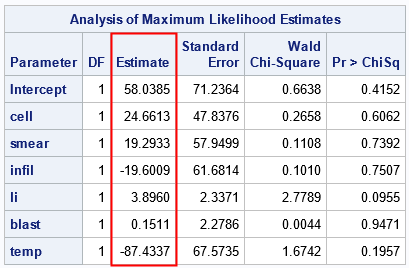The next section shows how to obtain the parameter estimates "manually" by performing a maximum-likelihood computation in the SAS/IML language.

### Logistic regression from scratch

Suppose you want to write a program to obtain the parameter estimates for this model. Notice that the regressors in the model are all continuous variables, and the model contains only main effects, which makes forming the design matrix easy. To fit a logistic model to data, you need to perform the following steps:

1. Read the data into a matrix and construct the design matrix by appending a column of 1s to represent the Intercept variable.
2. Write the loglikelihood function. This function will a vector of parameters (b) as input and evaluate the loglikelihood for the binary logistic model, given the data. The Penn State course in applied regression analysis explains the model and how to derive the loglikelihood function. The function is
$\ell(\beta) = \sum_{i=1}^{n}[y_{i}\log(\pi_{i})+(1-y_{i})\log(1-\pi_{i})]$
where $\pi_i$ is the i_th component of the logistic transformation of the linear predictor: $\pi(\beta) = \frac{1}{1+\exp(-X\beta)}$
3. Make an initial guess for the parameters and use nonlinear optimization to find the maximum likelihood estimates. The SAS/IML language supports several optimization methods. I use the Newton-Raphson method, which is implemented by using the NLPNRA subroutine.
proc iml; /* 1. read data and form design matrix */ varNames = {'cell' 'smear' 'infil' 'li' 'blast' 'temp'}; use remission; read all var "remiss" into y; /* read response variable */ read all var varNames into X; /* read explanatory variables */ close; X = j(nrow(X), 1, 1) || X; /* design matrix: add Intercept column */   /* 2. define loglikelihood function for binary logistic model */ start BinLogisticLL(b) global(X, y); z = X*b; /* X*b, where b is a column vector */ p = Logistic(z); /* 1 / (1+exp(-z)) */ LL = sum( y#log(p) + (1-y)#log(1-p) ); return( LL ); finish;   /* 3. Make initial guess and find parameters that maximize the loglikelihood */ b0 = j(1, ncol(X), 0); /* initial guess */ opt = 1; /* find maximum of function */ call nlpnra(rc, b, "BinLogisticLL", b0, opt); /* use Newton's method to find b that maximizes the LL function */ print b[c=("Intercept"||varNames) L="Parameter Estimates" F=D8.]; QUIT;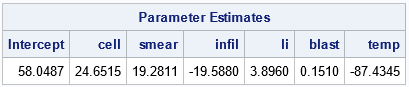The parameter estimates from the MLE are similar to the estimates from PROC LOGISTIC. When performing nonlinear optimization, different software uses different methods and criteria for convergence, so you will rarely get the exact parameter estimates when you solve the same problem by using different methods. For these data, the relative difference in each parameter estimate is ~1E-4 or less.

I didn't try to minimize the number of lines in the program. As written, the number of executable lines is 16. If you shorten the program by combining lines (for example, p = Logistic(X*b);), you can get the program down to 10 lines.

Of course, a program should never be judged solely by the number of lines it contains. I can convert any program to a "one-liner" by wrapping it in a macro! I prefer to ask whether the program mimics the mathematics underlying the analysis. Can the statements be understood by someone who is familiar with the underlying mathematics but might not be an expert programmer? Does the language provide analysts with the tools they need to perform and combine common operations in applied math and statistics? I find that the SAS/IML language succeeds in all these areas.

### Logistic regression with events-trials syntax

As mentioned previously, implementing the regression estimates "by hand" not only helps you to understand the problem better, but also to modify the basic program. For example, the syntax of PROC LOGISTIC enables you to analyze binomial data by using the events-trials syntax. When you have binomial data, each observation contains a variable (EVENTS) that indicates the number of successes and the total number of trials (TRIALS) for a specified value of the explanatory variables. The following DATA step defines data that are explained in the Getting Started documentation for PROC LOGISTIC. The data are for a designed experiment. For each combination of levels for the independent variables (HEAT and SOAK), each observation contains the number of items that were ready for further processing (R) out of the total number (N) of items that were tested. Thus, each observation represents N items for which R items are events (successes) and N-R are nonevents (failures).

The following DATA step defines the binomial data and calls PROC LOGISTIC to fit a logistic model:

/* Getting Started example for PROC LOGISTIC */ data ingots; input Heat Soak r n @@; datalines; 7 1.0 0 10 14 1.0 0 31 27 1.0 1 56 51 1.0 3 13 7 1.7 0 17 14 1.7 0 43 27 1.7 4 44 51 1.7 0 1 7 2.2 0 7 14 2.2 2 33 27 2.2 0 21 51 2.2 0 1 7 2.8 0 12 14 2.8 0 31 27 2.8 1 22 51 4.0 0 1 7 4.0 0 9 14 4.0 0 19 27 4.0 1 16 ;   proc logistic data=ingots; model r/n = Heat Soak; ods select ParameterEstimates; run;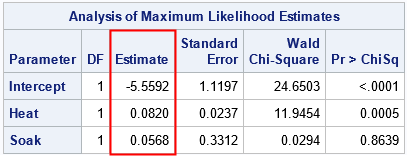Can you modify the previous SAS/IML program to accommodate binomial data? Yes! The key is "expand the data." Each observation represents N items for which R items are events (successes) and N-R are nonevents (failures). You could therefore reuse the previous program if you introduce a "frequency variable" (FREQ) that indicates the number of items that are represented by each observation.

The following program is a slight modification of the original program. The FREQ variable is derived from the EVENTS and TRIALS variables. The data is duplicated so that each observation in the binomial data becomes a pair of observations, one with frequency EVENTS and the other with frequency TRIALS-EVENTS. In the loglikelihood function, the frequency variable is incorporated into the summation.

proc iml; /* 1. read data and form design matrix */ varNames = {'Heat' 'Soak'}; use ingots; read all var "r" into Events; read all var "n" into Trials; read all var varNames into X; close; n = nrow(X); X = j(n,1,1) || X; /* design matrix: add Intercept column */   /* for event-trial syntax, split each data row into TWO observations, one for the events and one for the nonevents. Create Freq = frequency variable. */ Freq = Events // (Trials-Events); X = X // X; /* duplicate regressors */ y = j(n,1,1) // j(n,1,0); /* binary response: 1s and 0s */   /* 2. define loglikelihood function for events-trials logistic model */ start BinLogisticLLEvents(b) global(X, y, Freq); z = X*b; /* X*b, where b is a column vector */ p = Logistic(z); /* 1 / (1+exp(-z)) */ LL = sum( Freq#(y#log(p) + (1-y)#log(1-p)) ); /* count each observation numEvents times */ return( LL ); finish;   /* 3. Make initial guess and find parameters that maximize the loglikelihood */ b0 = j(1, ncol(X), 0); /* initial guess (row vector) */ opt = 1; /* find maximum of function */ call nlpnra(rc, b, "BinLogisticLLEvents", b0, opt); print b[c=("Intercept"||varNames) L="Parameter Estimates" F=D8.]; QUIT;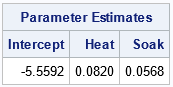For these data and model, the parameter estimates are the same to four decimal places. Again, there are small relative differences in the estimates. But the point is that you can solve for the MLE parameter estimates for binomial data from first principles by using about 20 lines of SAS/IML code.

### Summary

This article shows how to obtain parameter estimates for a binary regression model by writing about a short SAS/IML program. With slightly more effort, you can obtain parameter estimates for a binomial model in events-trials syntax. These examples demonstrate the power, flexibility, and compactness of the SAS/IML matrix programming language.

Of course, this power and flexibility come at a cost. When you use SAS/IML to solve a problem, you must understand the underlying mathematics of the problem. Second, the learning curve for SAS/IML is higher than for other SAS procedures. SAS procedures such as PROC LOGISTIC are designed so that you can focus on building a good predictive model without worrying about the details of numerical methods. Accordingly, SAS procedures can be used by analysts who want to focus solely on modeling. In contrast, the IML procedure is often used by sophisticated programmers who want to extend the capabilities of SAS by implementing custom algorithms.

The post Implement binary logistic regression from first principles appeared first on The DO Loop.

I previously wrote about partial leverage plots for regression diagnostics and why they are useful. You can generate a partial leverage plot in SAS by using the PLOTS=PARTIALPLOT option in PROC REG. One useful property of partial leverage plots is the ability to graphically represent the null hypothesis that a regression coefficient is 0. John Sall ("Leverage Plots for General Linear Hypotheses", TAS, 1990) showed that you could add a confidence band to the partial regression plot such that if the line segment for Y=0 is not completely inside the band, then you reject the null hypothesis.

This article shows two ways to get the confidence band. The easy way is to write the partial leverage data to a SAS data set and use the REG statement in PROC SGPLOT to overlay a regression fit and a confidence band for the mean. This way is easy to execute, but it requires performing an additional regression analysis for every effect in the model. A second way (which is due to Sall, 1990) computes the confidence band directly from the statistics on the original data. This article shows how to use the SAS/IML language to compute the confidence limits from the output of PROC REG.

### Confidence limits on a partial leverage plot: The easy way

The following example is taken from my previous article, which explains the code. The example uses a subset of Fisher's iris data. For convenience, the variables are renamed to Y (the response) and X1, X2, and X3 (the regressors). The call to PROC REG includes

data Have; set sashelp.iris( rename=(PetalLength = Y PetalWidth = x1 SepalLength = x2 SepalWidth = x3) where=(Species="Versicolor")); label Y= x1= x2= x3=; run;   proc reg data=Have plots(only)=PartialPlot; model Y = x1 x2 x3 / clb partial partialdata; ods exclude PartialPlotData; ods output PartialPlotData=PD; quit;   /* macro to create a partial leverage plot (with CLM) for each variable */ %macro MakePartialPlot(VarName); title "Partial Leverage Plot for &VarName"; title2 "with 95% CL for H0: Beta_&VarName = 0"; proc sgplot data=PD ; reg y=part_Y_&VarName x=part_&VarName / CLM; refline 0 / axis=y; run; %mend;   %MakePartialPlot(x2); %MakePartialPlot(x3);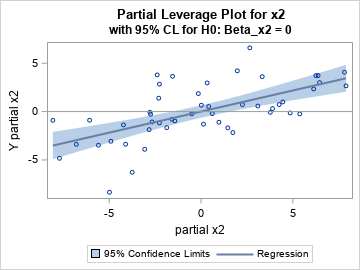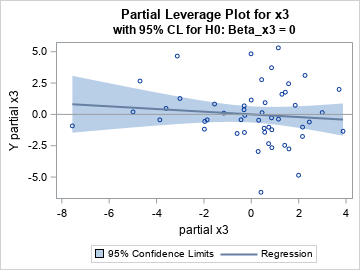### Confidence limits on a partial leverage plot: The efficient way

The previous section shows an easy way to produce partial leverage regression plots in SAS. The confidence bands are a graphical way to examine the null hypotheses βi = 0. Look at the confidence band for the partial leverage plot for the X2 variable. The Y=0 axis (the gray line) is NOT wholly contained in the confidence band. You can therefore reject (with 95% confidence) the null hypotheses that β2=0. In contrast, look at the confidence band for the partial leverage plot for the X3 variable. The Y=0 axis IS wholly contained in the confidence band. You therefore cannot reject the null hypotheses that β3=0.

Although this method is easy, Sall (1990) points out that it is not the most computationally efficient method. For every variable, the SGPLOT procedure computes a bivariate regression, which means that this method requires performing k+2 linear regressions: the regression for the original data that has k regressors, followed by additional bivariate regression for the intercept and each of the k regressors. In practice, each bivariate regression can be computed very quickly, so the entire process is not very demanding. Nevertheless, Sall (1990) showed that all the information you need is already available from the regression on the original data. You just need to save that information and use it to construct the confidence bands.

The following section uses Sall's method to compute the quantities for the partial leverage plots. The program is included for the mathematically curious; I do not recommend that you use it in practice because the previous method is much simpler.

### Sall's method for computing partial leverage regression plots

Sall's method requires the following output from the original regression model:

• The means of the regressor variables. You can get these statistics by using the SIMPLE option on the PROC REG statement and by using ODS OUTPUT SimpleStatistics=Simple to save the statistics to a data set.
• The inverse of the crossproduct matrix (the X*X matrix). You can get this matrix by using the I option on the MODEL statement and by using ODS OUTPUT InvXPX=InvXPX to save the matrix to a data set.
• The mean square error and the error degrees of freedom for the regression model. You can get these statistics by using ODS OUTPUT ANOVA=Anova to save the ANOVA table to a data set.
• The parameter estimates and the F statistics for the null hypotheses βi=0. You can get these statistics by using the CLB option on the MODEL statement and by using ODS OUTPUT ParameterEstimates=PE to save the parameter estimates table to a data set. The data set contains t statistics for the null hypotheses, but the F statistic is the square of the t statistic.

The following call to PROC REG requests all the statistics and saves them to data sets:

proc reg data=Have plots(only)=PartialPlot simple; model Y = x1 x2 x3 / clb partial partialdata I; ods exclude InvXPX PartialPlotData; ods output SimpleStatistics=Simple InvXPX=InvXPX ANOVA=Anova ParameterEstimates=PE PartialPlotData=PD(drop=Model Dependent Observation); quit;

Sall (1990) shows how to use these statistics to create the partial leverage plots. The following SAS/IML program implements Sall's method.

/* Add confidence bands to partial leverage plots: Sall, J. P. (1990). "Leverage Plots for General Linear Hypotheses." American Statistician 44:308–315. */ proc iml; alpha = 0.05;   /* Use statistics from original data to estimate the confidence bands */ /* xBar = mean of each original variable */ use Simple; read all var "Mean" into xBar; close; p = nrow(xBar)-1; /* number of parameters */ xBar = xBar[1:p]; /* remove the Y mean */   /* get (X*X)^{-1} matrix */ use InvXPX; read all var _NUM_ into xpxi; close; xpxi = xpxi[1:p, 1:p]; /* remove column and row for Y */ hbar = xbar * xpxi * xbar; /* compute hbar from inv(X*X) and xbar */   /* get s2 = mean square error and error DF */ use ANOVA; read all var {DF MS}; close; s2 = MS; /* extract MSE */ DFerror = DF; /* extract error DF */   /* get parameter estimates and t values */ use PE; read all var {Estimate tValue}; close; FValue = tValue#tValue; /* F statistic is t^2 for OLS */ b = Estimate; /* lines have slope b[i] and intercept 0 */   tAlpha = quantile("T", 1-alpha/2, DFerror); /* assume DFerror > 0 */ FAlpha = tAlpha#tAlpha; /* = quantile( "F", 1-alpha, 1, DFerror); */   /* Get partial plot data from REG output */ /* read in part_: names for intercept and X var names */ use PD; read all var _NUM_ into partial[c=partNames]; close;   /* The formula for the confidence limits is from Sall (1990) */ nPts = 100; /* score CL at nPts values on [xMin, xMax] */ XPts = j(nPts, p, .); /* allocate matrices to store the results */ Pred = j(nPts, p, .); Lower = j(nPts, p, .); Upper = j(nPts, p, .); do i = 1 to p; levX = partial[,2*i-1]; /* partial x variable */ xMin = min(levX); xMax = max(levX); /* [xMin, xMax] */ /* partial regression line is (t,z) and CL band is (t, z+/- SE) */ t = T( do(xMin,xMax,(xMax-xMin)/(nPts-1))); /* assume xMin < xMax */ z = b[i]*t; /* predicted mean: yHat = b[i]*x + 0 */ SE = sqrt( s2#FAlpha#hbar + FAlpha/FValue[i] # (z#z) ); /* Sall, 1990 */ XPts[,i] = t; Pred[,i] = z; Lower[,i] = z - SE; Upper[,i] = z + SE; end;   /* create names for new variables for the CL band */ oddIdx = do(1,ncol(partNames),2); /* names we want are the ODD elements */ Names = partNames[,oddIdx]; XNames = compress('X_' +Names); PredNames = compress('Pred_'+Names); LowerNames = compress('LCL_' +Names); UpperNames = compress('UCL_' +Names);   /* write regression lines and confidence bands to a data set */ Results = XPts || Pred || Lower || Upper; create PartCL from Results[c=(XNames||PredNames||LowerNames||UpperNames)]; append from Results; close; QUIT;

The data set PartCL contains the fit lines (which have slopes equal to b[i]) and the lower and upper confidence limits for the means of the partial leverage plots. The lines are evaluated at 100 evenly spaced points in the range of the "partial variables." The program uses matrix multiplication to evaluate the quadratic form $\bar{x}^\prime (X^\prime X)^{-1} \bar{x}$. The remaining computations are scalar operations.

The following statements merge the regression lines and confidence intervals with the data for the partial leverage plots. You can then create a scatter plot of the partial leverage values and overlay the regression line and confidence bands.

data Want; set PD PartCL; run;   /* macro for plotting a partial leverage plot with CL from the IML program */ %macro MakePartialPlotCL(VarName); title "Partial Leverage Plot for &VarName"; proc sgplot data=Want noautolegend; band x=x_part_&VarName lower=LCL_part_&VarName upper=UCL_part_&VarName; scatter x=part_&VarName y=part_Y_&VarName; series x=x_part_&VarName y=Pred_part_&VarName; refline 0 / axis=y; run; %mend;   ods graphics / PUSH AttrPriority=None width=360px height=270px; %MakePartialPlotCL(x2); %MakePartialPlotCL(x3); ods graphics / POP; /* restore ODS GRAPHICS options */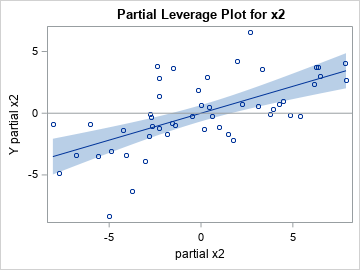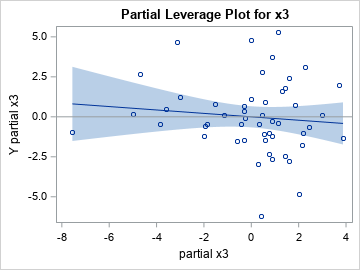As expected, the graphs are the same as the ones in the previous section. However, the quantities in the graphs are all produced by PROC REG as part of the computations for the original regression model. No additional regression computations are required.

### Summary

This article shows two ways to get a confidence band for the conditional mean of a partial regression plot. The easy way is to use the REG statement in PROC SGPLOT. A more efficient way is to compute the confidence band directly from the statistics on the original data. This article shows how to use the SAS/IML language to compute the confidence limits from the output of PROC REG.

This article also demonstrates a key reason why SAS customers choose SAS/IML software. They want to compute some statistic that is not directly produced by any SAS procedure. The SAS/IML language enables you to combine the results of SAS procedures and use matrix operations to compute other statistics from formulas in textbooks or journal articles. In this case, PROC IML consumes the output of PROC REG and creates regression lines and confidence bands for partial leverage plots.

The post Confidence bands for partial leverage regression plots appeared first on The DO Loop.

M estimation is a robust regression technique that assigns a weight to each observation based on the magnitude of the residual for that observation. Large residuals are downweighted (assigned weights less than 1) whereas observations with small residuals are given weights close to 1. By iterating the reweighting and fitting steps, the method converges to a regression that de-emphasizes observations whose residuals are much bigger than the others. This iterative process is also known called iteratively reweighted least squares.

A previous article visualizes 10 different "weighting functions" that are supported by the ROBUSTREG procedure in SAS. That article mentions that the results of the M estimation process depend on the choice of the weighting function. This article runs three robust regression models on the same data. The only difference between the three models is the choice of a weighting function. By comparing the regression estimates for each model, you can understand how the choice of a weighting function affects the final model.

### Data for robust regression

The book Applied Regression Analysis and Generalized Linear Models (3rd Ed, J. Fox, 2016) analyzes Duncan's occupational prestige data (O. D. Duncan, 1961), which relates prestige to the education and income of men in 45 occupations in the US in 1950. The following three variables are in the data set:

• Income (Explanatory): Percent of males in the occupation who earn $3,500 or more. • Education (Explanatory): Percent of males in the occupation who are high-school graduates. • Prestige (Response): Percent of raters who rated the prestige of the occupation as excellent or good. The following scatter plot shows the distributions of the Income and Education variables. The color of the marker reflects the Prestige variable. Certain occupations are labeled: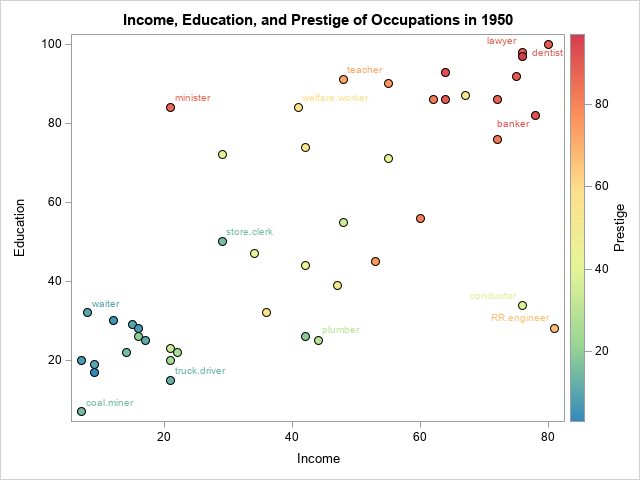The markers are roughly divided into four regions: • The cluster of markers in the lower-left corner of the graph are unskilled laborers such as coal miners, truck drivers, and waiters. The men in these occupations have little education and earn little income. • Markers in the lower-right corner represent are skilled laborers such as plumbers and railroad conductors who make considerable income despite having little education. • Markers in the upper-left corner represent occupations such as ministers and welfare workers who have considerable education but low-paying jobs. • Markers in the upper-right corner represent occupations such as doctors, lawyers, and bankers who have a lot of education and high income. In general, the prestige of an occupation increases from lower-left to upper-right. However, there are some exceptions. A minister has more prestige than might be expected from his income. Reporters and railroad conductors have less prestige than you might expect from their income. A robust regression model that uses M estimation will assign smaller weights to observations that do not match the major trends in the data. Observations that have very small weights are classified as outliers. The following SAS DATA step creates Duncan's prestige data: /* Duncan's Occupational Prestige Data from John Fox (2016), Applied Regression Analysis and Generalized Linear Models, Third Edition https://socialsciences.mcmaster.ca/jfox/Books/Applied-Regression-3E/datasets/Duncan.txt Original source: Duncan, O. D. (1961) A socioeconomic index for all occupations. In Reiss, A. J., Jr. (Ed.) Occupations and Social Status. Free Press [Table VI-1]. Type: prof=Professional, wc=White collar, bc=Blue collar */ data Duncan; length Occupation$20 Type $4; input Occupation Type Income Education Prestige; ObsNum + 1; datalines; accountant prof 62 86 82 pilot prof 72 76 83 architect prof 75 92 90 author prof 55 90 76 chemist prof 64 86 90 minister prof 21 84 87 professor prof 64 93 93 dentist prof 80 100 90 reporter wc 67 87 52 engineer prof 72 86 88 undertaker prof 42 74 57 lawyer prof 76 98 89 physician prof 76 97 97 welfare.worker prof 41 84 59 teacher prof 48 91 73 conductor wc 76 34 38 contractor prof 53 45 76 factory.owner prof 60 56 81 store.manager prof 42 44 45 banker prof 78 82 92 bookkeeper wc 29 72 39 mail.carrier wc 48 55 34 insurance.agent wc 55 71 41 store.clerk wc 29 50 16 carpenter bc 21 23 33 electrician bc 47 39 53 RR.engineer bc 81 28 67 machinist bc 36 32 57 auto.repairman bc 22 22 26 plumber bc 44 25 29 gas.stn.attendant bc 15 29 10 coal.miner bc 7 7 15 streetcar.motorman bc 42 26 19 taxi.driver bc 9 19 10 truck.driver bc 21 15 13 machine.operator bc 21 20 24 barber bc 16 26 20 bartender bc 16 28 7 shoe.shiner bc 9 17 3 cook bc 14 22 16 soda.clerk bc 12 30 6 watchman bc 17 25 11 janitor bc 7 20 8 policeman bc 34 47 41 waiter bc 8 32 10 ; ### Analysis 1: The bisquare weighting function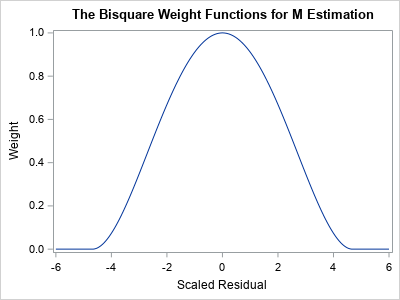The following call to PROC ROBUSTREG shows how to run a robust regression analysis of the Duncan prestige data. The METHOD=M option tells the procedure to use M estimation. The WEIGHTFUNCTION= suboption specifies the weight function that will assign weights to observations based on the size of the residuals. The default weight function is the bisquare function, but the following statements specify the weight function explicitly. A graph of the bisquare weighting function is shown to the right. Observations that have a small residual receive a weight near 1; outliers receive a weight close to 0. title "Robust Regression Analysis for WEIGHTFUNCTION=Bisquare"; proc robustreg data=Duncan plots=RDPlot method=M(WEIGHTFUNCTION=Bisquare); model Prestige = Income Education; ID Occupation; output out=RROut SResidual=StdResid Weight=Weight Leverage=Lev Outlier=Outlier; ods select ParameterEstimates RDPlot; run;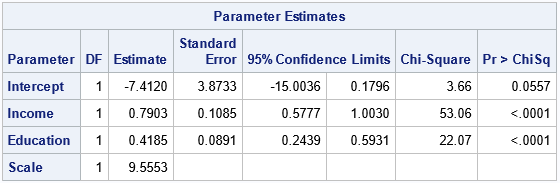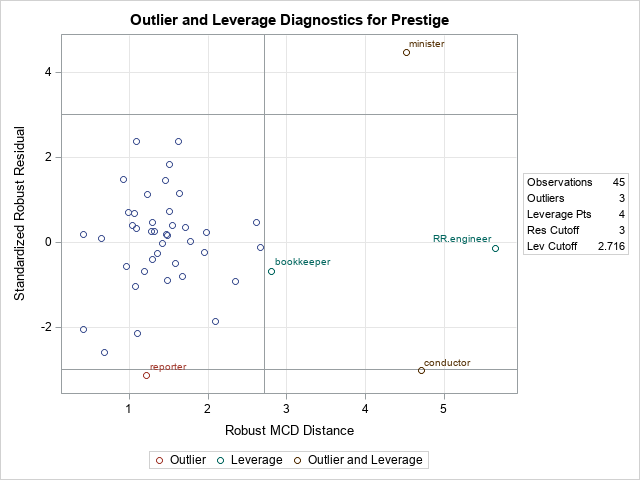The parameter estimates are shown. The bisquare-weighted regression model is Prestige = -7.41 + 0.79*Income + 0.42*Education. The intercept term is not significant at the 0.05 significance level, so you could rerun the analysis to use a no-intercept model. We will not do that here. The plot is called the residual-distance plot (RD plot). You can use an RD plot to identify high-leverage points and outliers for the model. This is a robust version of the residual-leverage plot that is used for ordinary least-squares regression. • The horizontal direction is a robust measure of the leverage. Think of it as a robust distance from the coordinates of each observation to a robust estimate of the center of the explanatory variables. Observations that have a large distance are high-leverage points. M estimation is not robust to the presence of high-leverage points, so SAS will display a WARNING in the log that states "The data set contains one or more high-leverage points, for which M estimation is not robust." You can see from this plot (or from the scatter plot in the previous section) that bookkeepers, ministers, conductors, and railroad engineers are somewhat far from the center of the point cloud in the bivariate space of the explanatory variables (Income and Education). Therefore, they are high-leverage points. • The vertical direction shows the size of a standardized residual for the robust regression model. Reference lines are drawn at ±3. If an observation has a residual that exceeds these values, it is considered an outlier, meaning that the value of Prestige in the data is much higher or lower than the value predicted by the model. You can see from this plot that ministers, reporters, and conductors are outliers for this model. The graph of the bisquare weighting function enables you to mentally estimate the weights for each observation. But an easier way to visualize the weights is to use the WEIGHT= option on the OUTPUT statement to output the weights to a SAS data set. You can then plot the weight for each observation and label the markers that have small weights, as follows: data RROut2; set RROut; length Label$20; if Weight < 0.8 then Label = Occupation; else Label = ""; run;   title "Weights for WEIGHTFUNCTION=Bisquare"; proc sgplot data=RROut2; scatter x=ObsNum y=Weight / datalabel=Label; run;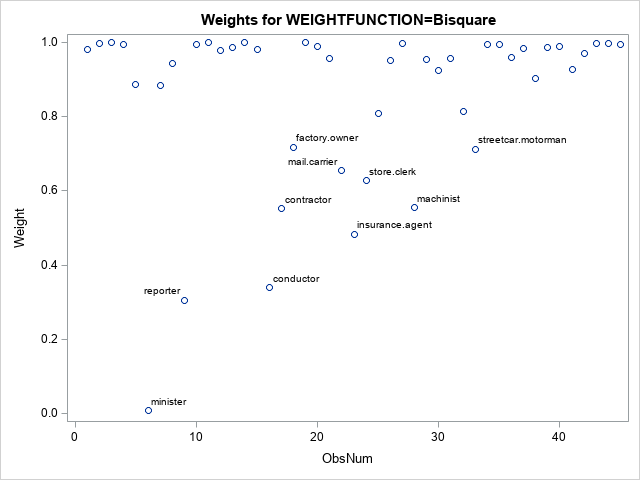The scatter plot (click to enlarge) shows the weights for all observations. A few that have small weights (including the outliers) are labeled. You can see that the minister has a weight close to Weight=0 whereas reporters and conductors have weights close to Weight=0.3. These weights are used in a weighted regression, which is the final regression model.

If you prefer a tabular output, you can use the LEVERAGE= and OUTLIER= options on the OUTPUT statement to output indicator variables that identify each observation as a high-leverage point, an outlier, or both. The following call to PROC PRINT shows how to display only the outliers and high-leverage points:

proc print data=RROut; where Lev=1 | Outlier=1; var Occupation Weight Prestige Income Education Lev Outlier; run;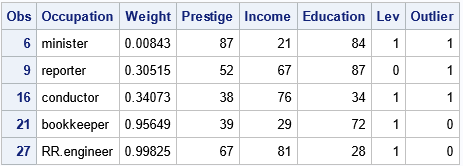The output shows that four observations are high-leverage points and three are outliers. (The minister and reporter occupations are classified as both.) This outlier classification depends on the weighting function that you use during the M estimation procedure.

### Create a SAS macro to repeat the analysis

You can repeat the previous analysis for other weighting functions to see how the choice of a weighting function affects the final regression fit. It makes sense to wrap up the main functionality into a SAS macro that takes the name of the weighting function as an argument, as follows:

%macro RReg( WeightFunc ); title "Robust Regression Analysis for WEIGHTFUNCTION=&WeightFunc"; proc robustreg data=Duncan plots=RDPlot method=M(WEIGHTFUNCTION=&WeightFunc); model Prestige = Income Education; ID Occupation; output out=RROut SResidual=StdResid Weight=Weight; ods select ParameterEstimates RDPlot; run;   data RROut2; set RROut; length Label $20; if Weight < 0.8 then Label = Occupation; else Label = ""; run; title "Weights for WEIGHTFUNCTION=&WeightFunc"; proc sgplot data=RROut2; scatter x=ObsNum y=Weight / datalabel=Label; run; %mend; The next sections call the macro for the Huber and Talworth weighting functions, but you can use it for any of the 10 weighting functions that PROC ROBUSTREG supports. ### Analysis 2: The Huber weighting function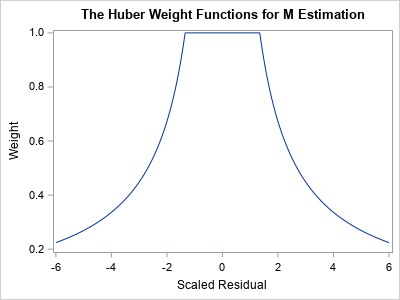A graph of the Huber weight function is shown to the right. Notice that the any observation whose standardized residual is less than 1.345 receives a weight of 1. Observations that have larger residuals are downweighted by using a weight that is inversely proportional to the magnitude of the residual. The following statement re-runs the M estimation analysis, but uses the Huber weighting function: %RReg( Huber ); The parameter estimates are not shown, but the Huber-weighted regression model is Prestige = -7.1107 + 0.70*Income + 0.49*Education. Notice that the estimates are different from the bisquare analysis because the Huber and bisquare functions result in different weights. For this model, reporters and ministers are outliers. Many observations receive full weight (Weight=1) in the analysis. A handful of observations are downweighted, as shown in the following scatter plot (click to enlarge):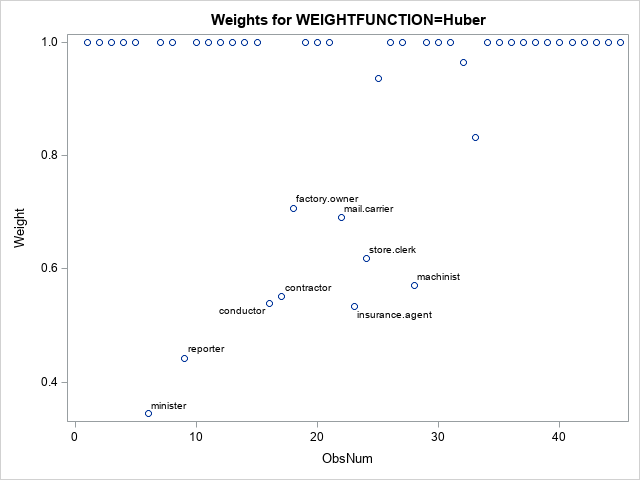### Analysis 3: The Talworth weighting function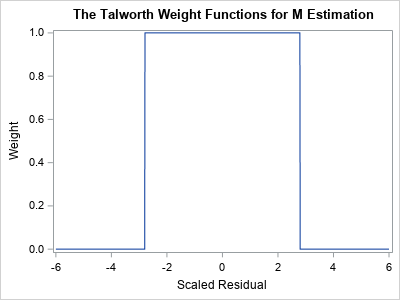A graph of the Talworth weight function is shown to the right. Notice that any observation whose standardized residual is less than 2.795 receives a weight of 1. All other observations are excluded from the analysis by assigning them a weight of 0. The following statement re-runs the M estimation analysis, but uses the Talworth weighting function: %RReg( Talworth ); The parameter estimates are not shown, but the Talworth-weighted regression model is Prestige = -7.45 + 0.74*Income + 0.46*Education. Again, the estimates are different from the estimates that used the other weighting functions. For this analysis, the reporters and ministers are outliers. All other observations receive Weight=1. The weights are shown in the following scatter plot (click to enlarge):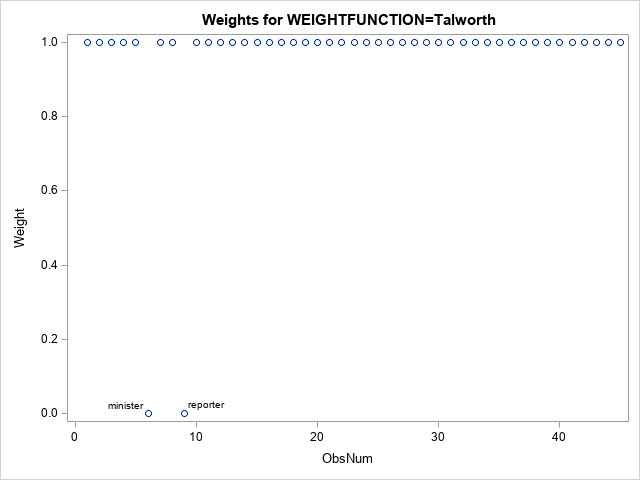### Summary Many statistical procedures depend on parameters, which affect the results of the procedure. Users of SAS software often accept the default methods, but it is important to recognize that an analysis can and will change if you use different parameters. For the ROBUSTREG procedure, the default method is M estimation, and the default weighting function is the bisquare weighting function. This article demonstrates that the fitted regression model will change if you use a different weighting function. Different weighting functions can affect which observations are classified as outliers. Each function assigns different weights to observations that have large residual values. The post The effect of weight functions in a robust regression method appeared first on The DO Loop. M estimation is a robust regression technique that assigns a weight to each observation based on the magnitude of the residual for that observation. Large residuals are downweighted (assigned weights less than 1) whereas observations with small residuals are given weights close to 1. By iterating the reweighting and fitting steps, the method converges to a regression that de-emphasizes observations whose residuals are much bigger than the others. This iterative process is also known called iteratively reweighted least squares. A previous article visualizes 10 different "weighting functions" that are supported by the ROBUSTREG procedure in SAS. That article mentions that the results of the M estimation process depend on the choice of the weighting function. This article runs three robust regression models on the same data. The only difference between the three models is the choice of a weighting function. By comparing the regression estimates for each model, you can understand how the choice of a weighting function affects the final model. ### Data for robust regression The book Applied Regression Analysis and Generalized Linear Models (3rd Ed, J. Fox, 2016) analyzes Duncan's occupational prestige data (O. D. Duncan, 1961), which relates prestige to the education and income of men in 45 occupations in the US in 1950. The following three variables are in the data set: • Income (Explanatory): Percent of males in the occupation who earn$3,500 or more.
• Education (Explanatory): Percent of males in the occupation who are high-school graduates.
• Prestige (Response): Percent of raters who rated the prestige of the occupation as excellent or good.

The following scatter plot shows the distributions of the Income and Education variables. The color of the marker reflects the Prestige variable. Certain occupations are labeled:The markers are roughly divided into four regions:

• The cluster of markers in the lower-left corner of the graph are unskilled laborers such as coal miners, truck drivers, and waiters. The men in these occupations have little education and earn little income.
• Markers in the lower-right corner represent are skilled laborers such as plumbers and railroad conductors who make considerable income despite having little education.
• Markers in the upper-left corner represent occupations such as ministers and welfare workers who have considerable education but low-paying jobs.
• Markers in the upper-right corner represent occupations such as doctors, lawyers, and bankers who have a lot of education and high income.

In general, the prestige of an occupation increases from lower-left to upper-right. However, there are some exceptions. A minister has more prestige than might be expected from his income. Reporters and railroad conductors have less prestige than you might expect from their income. A robust regression model that uses M estimation will assign smaller weights to observations that do not match the major trends in the data. Observations that have very small weights are classified as outliers.

The following SAS DATA step creates Duncan's prestige data:

/* Duncan's Occupational Prestige Data from John Fox (2016), Applied Regression Analysis and Generalized Linear Models, Third Edition https://socialsciences.mcmaster.ca/jfox/Books/Applied-Regression-3E/datasets/Duncan.txt Original source: Duncan, O. D. (1961) A socioeconomic index for all occupations. In Reiss, A. J., Jr. (Ed.) Occupations and Social Status. Free Press [Table VI-1].   Type: prof=Professional, wc=White collar, bc=Blue collar */ data Duncan; length Occupation $20 Type$4; input Occupation Type Income Education Prestige; ObsNum + 1; datalines; accountant prof 62 86 82 pilot prof 72 76 83 architect prof 75 92 90 author prof 55 90 76 chemist prof 64 86 90 minister prof 21 84 87 professor prof 64 93 93 dentist prof 80 100 90 reporter wc 67 87 52 engineer prof 72 86 88 undertaker prof 42 74 57 lawyer prof 76 98 89 physician prof 76 97 97 welfare.worker prof 41 84 59 teacher prof 48 91 73 conductor wc 76 34 38 contractor prof 53 45 76 factory.owner prof 60 56 81 store.manager prof 42 44 45 banker prof 78 82 92 bookkeeper wc 29 72 39 mail.carrier wc 48 55 34 insurance.agent wc 55 71 41 store.clerk wc 29 50 16 carpenter bc 21 23 33 electrician bc 47 39 53 RR.engineer bc 81 28 67 machinist bc 36 32 57 auto.repairman bc 22 22 26 plumber bc 44 25 29 gas.stn.attendant bc 15 29 10 coal.miner bc 7 7 15 streetcar.motorman bc 42 26 19 taxi.driver bc 9 19 10 truck.driver bc 21 15 13 machine.operator bc 21 20 24 barber bc 16 26 20 bartender bc 16 28 7 shoe.shiner bc 9 17 3 cook bc 14 22 16 soda.clerk bc 12 30 6 watchman bc 17 25 11 janitor bc 7 20 8 policeman bc 34 47 41 waiter bc 8 32 10 ;

### Analysis 1: The bisquare weighting functionThe following call to PROC ROBUSTREG shows how to run a robust regression analysis of the Duncan prestige data. The METHOD=M option tells the procedure to use M estimation. The WEIGHTFUNCTION= suboption specifies the weight function that will assign weights to observations based on the size of the residuals.

The default weight function is the bisquare function, but the following statements specify the weight function explicitly. A graph of the bisquare weighting function is shown to the right. Observations that have a small residual receive a weight near 1; outliers receive a weight close to 0.

title "Robust Regression Analysis for WEIGHTFUNCTION=Bisquare"; proc robustreg data=Duncan plots=RDPlot method=M(WEIGHTFUNCTION=Bisquare); model Prestige = Income Education; ID Occupation; output out=RROut SResidual=StdResid Weight=Weight Leverage=Lev Outlier=Outlier; ods select ParameterEstimates RDPlot; run;The parameter estimates are shown. The bisquare-weighted regression model is
Prestige = -7.41 + 0.79*Income + 0.42*Education.
The intercept term is not significant at the 0.05 significance level, so you could rerun the analysis to use a no-intercept model. We will not do that here.

The plot is called the residual-distance plot (RD plot). You can use an RD plot to identify high-leverage points and outliers for the model. This is a robust version of the residual-leverage plot that is used for ordinary least-squares regression.

• The horizontal direction is a robust measure of the leverage. Think of it as a robust distance from the coordinates of each observation to a robust estimate of the center of the explanatory variables. Observations that have a large distance are high-leverage points. M estimation is not robust to the presence of high-leverage points, so SAS will display a WARNING in the log that states "The data set contains one or more high-leverage points, for which M estimation is not robust." You can see from this plot (or from the scatter plot in the previous section) that bookkeepers, ministers, conductors, and railroad engineers are somewhat far from the center of the point cloud in the bivariate space of the explanatory variables (Income and Education). Therefore, they are high-leverage points.
• The vertical direction shows the size of a standardized residual for the robust regression model. Reference lines are drawn at ±3. If an observation has a residual that exceeds these values, it is considered an outlier, meaning that the value of Prestige in the data is much higher or lower than the value predicted by the model. You can see from this plot that ministers, reporters, and conductors are outliers for this model.

The graph of the bisquare weighting function enables you to mentally estimate the weights for each observation. But an easier way to visualize the weights is to use the WEIGHT= option on the OUTPUT statement to output the weights to a SAS data set. You can then plot the weight for each observation and label the markers that have small weights, as follows:

data RROut2; set RROut; length Label $20; if Weight < 0.8 then Label = Occupation; else Label = ""; run; title "Weights for WEIGHTFUNCTION=Bisquare"; proc sgplot data=RROut2; scatter x=ObsNum y=Weight / datalabel=Label; run;The scatter plot (click to enlarge) shows the weights for all observations. A few that have small weights (including the outliers) are labeled. You can see that the minister has a weight close to Weight=0 whereas reporters and conductors have weights close to Weight=0.3. These weights are used in a weighted regression, which is the final regression model. If you prefer a tabular output, you can use the LEVERAGE= and OUTLIER= options on the OUTPUT statement to output indicator variables that identify each observation as a high-leverage point, an outlier, or both. The following call to PROC PRINT shows how to display only the outliers and high-leverage points: proc print data=RROut; where Lev=1 | Outlier=1; var Occupation Weight Prestige Income Education Lev Outlier; run;The output shows that four observations are high-leverage points and three are outliers. (The minister and reporter occupations are classified as both.) This outlier classification depends on the weighting function that you use during the M estimation procedure. ### Create a SAS macro to repeat the analysis You can repeat the previous analysis for other weighting functions to see how the choice of a weighting function affects the final regression fit. It makes sense to wrap up the main functionality into a SAS macro that takes the name of the weighting function as an argument, as follows: %macro RReg( WeightFunc ); title "Robust Regression Analysis for WEIGHTFUNCTION=&WeightFunc"; proc robustreg data=Duncan plots=RDPlot method=M(WEIGHTFUNCTION=&WeightFunc); model Prestige = Income Education; ID Occupation; output out=RROut SResidual=StdResid Weight=Weight; ods select ParameterEstimates RDPlot; run; data RROut2; set RROut; length Label$20; if Weight < 0.8 then Label = Occupation; else Label = ""; run;   title "Weights for WEIGHTFUNCTION=&WeightFunc"; proc sgplot data=RROut2; scatter x=ObsNum y=Weight / datalabel=Label; run; %mend;

The next sections call the macro for the Huber and Talworth weighting functions, but you can use it for any of the 10 weighting functions that PROC ROBUSTREG supports.

### Analysis 2: The Huber weighting functionA graph of the Huber weight function is shown to the right. Notice that the any observation whose standardized residual is less than 1.345 receives a weight of 1. Observations that have larger residuals are downweighted by using a weight that is inversely proportional to the magnitude of the residual.

The following statement re-runs the M estimation analysis, but uses the Huber weighting function:

%RReg( Huber );

The parameter estimates are not shown, but the Huber-weighted regression model is
Prestige = -7.1107 + 0.70*Income + 0.49*Education.
Notice that the estimates are different from the bisquare analysis because the Huber and bisquare functions result in different weights.

For this model, reporters and ministers are outliers. Many observations receive full weight (Weight=1) in the analysis. A handful of observations are downweighted, as shown in the following scatter plot (click to enlarge):### Analysis 3: The Talworth weighting functionA graph of the Talworth weight function is shown to the right. Notice that any observation whose standardized residual is less than 2.795 receives a weight of 1. All other observations are excluded from the analysis by assigning them a weight of 0.

The following statement re-runs the M estimation analysis, but uses the Talworth weighting function:

%RReg( Talworth );

The parameter estimates are not shown, but the Talworth-weighted regression model is
Prestige = -7.45 + 0.74*Income + 0.46*Education.
Again, the estimates are different from the estimates that used the other weighting functions.

For this analysis, the reporters and ministers are outliers. All other observations receive Weight=1. The weights are shown in the following scatter plot (click to enlarge):### Summary

Many statistical procedures depend on parameters, which affect the results of the procedure. Users of SAS software often accept the default methods, but it is important to recognize that an analysis can and will change if you use different parameters. For the ROBUSTREG procedure, the default method is M estimation, and the default weighting function is the bisquare weighting function. This article demonstrates that the fitted regression model will change if you use a different weighting function. Different weighting functions can affect which observations are classified as outliers. Each function assigns different weights to observations that have large residual values.

The post The effect of weight functions in a robust regression method appeared first on The DO Loop.

An early method for robust regression was iteratively reweighted least-squares regression (Huber, 1964). This is an iterative procedure in which each observation is assigned a weight. Initially, all weights are 1. The method fits a least-squares model to the weighted data and uses the size of the residuals to determine a new set of weights for each observation. In most implementations, observations that have large residuals are downweighted (assigned weights less than 1) whereas observations with small residuals are given weights close to 1. By iterating the reweighting and fitting steps, the method converges to a regression that de-emphasizes observations whose residuals are much bigger than the others.

This form of robust regression is also called M estimation. The "M" signifies that the method is similar to Maximum likelihood estimation. The method tries to minimize a function of the weighted residuals. This method is not robust to high-leverage points because they have a disproportionate influence on the initial fit, which often means they have small residuals and are never downweighted.

The results of the regression depend on the function that you use to weight the residuals. The ROBUSTREG procedure in SAS supports 10 different "weighting functions." Some of these assign a zero weight to observations that have large residuals, which essentially excludes that observation from the fit. Others use a geometric or exponentially decaying function to assign small (but nonzero) weights to large residual values. This article visualizes the 10 weighting functions that are available in SAS software for performing M estimation in the ROBUSTREG procedure. You can use the WEIGHTFUNCTION= suboption to the METHOD=M option on the PROC ROBUSTREG statement to choose a weighting function.

See the documentation of the ROBUSTREG procedure for the formulas and default parameter values for the 10 weighting functions. The residuals are standardized by using a robust estimate of scale. In the rest of this article, "the residual" actually refers to a standardized residual, not the raw residual. The plots of the weighting functions are shown on the interval[-6, 6] and show how functions assign weights based on the magnitude of the standardized residuals.

### Differentiable weighting functions

If you are using iteratively reweighted least squares to compute the estimates, it doesn't matter whether the weighting functions are differentiable. However, if you want to use optimization methods to solve for the parameter estimates, many popular optimization algorithms work best when the objective function is differentiable. The objective function is often related to a sum that involves the weighted residuals, so let's first look at weighting functions that are differentiable. The following four functions are differentiable transformations that assign weights to observations based on the size of the standardized residual.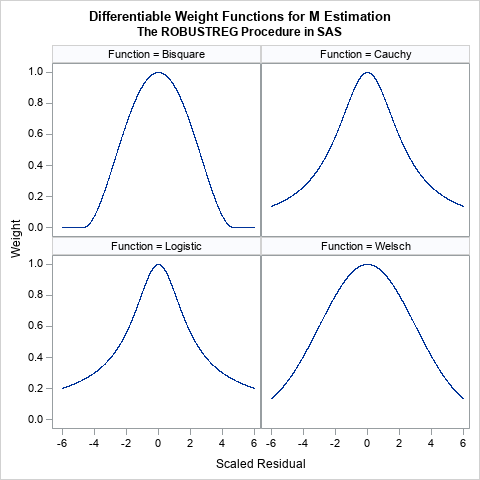These functions all look similar. They are all symmetric functions and look like the quadratic function 1 – a r2 close to r = 0. However, the functions differ in the rate at which they decrease away from r = 0:

• The bisquare function (also called the Tukey function) is a quartic function on the range [-c, c] and equals 0 outside that interval. This is the default weight function for M estimation in PROC ROBUSTREG. The function is chosen so that the derivative at ±c is 0, and thus the function is differentiable. An observation is excluded from the computation if the magnitude of the standardized residual exceeds c. By default, c=4.685.
• The Cauchy and Logistic functions are smooth functions that decrease exponentially. If you use these functions, the weight for an observation is never 0, but the weight falls off rapidly as the magnitude of r increases.
• The Welsch function is a rescaled Gaussian function. The weight for an observation is never 0, but the weight falls off rapidly (like exp(-a r2)) as the magnitude of r increases.

### Nondifferentiable weighting functions

The following six functions are not differentiable at one or more points.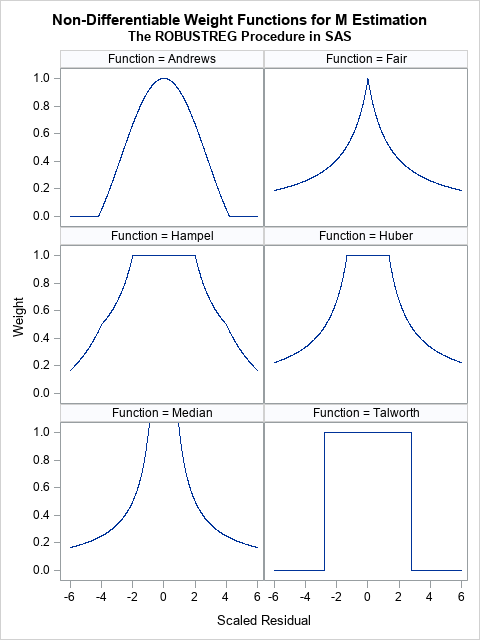• The Andrews function is the function c*sin(r/c)/r within the interval [-c π, c π] and 0 outside. a quartic function on the range [-c, c] and equals 0 outside that interval. It is similar to the bisquare function (see the previous section) but is not differentiable at ±c π.
• The Fair and Median functions are similar. The assign a weight that is asymptotically proportional to 1/|r|. Use the Fair function if you want to downweight observations according to the inverse magnitude of their residual value. Do not use the Median function, which assigns huge weights to observations with small residuals.
• The Hampel, Huber, and Talworth functions are similar because they all assign unit weight to residuals that are small in magnitude. The Talworth function is the most Draconian weight function: it assigns each observation a weight of 1 or 0, depending on whether the magnitude of the residual exceeds some threshold. The Hampel and Huber functions assign a weight of 1 for small residuals, then assign weights in a decreasing manner. If you use the default parameter values for the Hampel function, the weights are exactly 0 outside of the interval [-8, 8].

When I use M estimation, I typically use one of the following three functions:

• The bisquare function, which excludes observations whose residuals have a magnitude greater than 4.685.
• The Welsch function, which gently downweights large residuals.
• The Huber function, which assigns 1 for the weight of small residuals and assigns weights to larger residuals that are proportional to the inverse magnitude.

### Summary

M estimation is a robust regression technique that assigns weights to each observation. The idea is to assign small (or even zero) weights to observations that have large residuals and weights that are close to unity for residuals that are small. (In practice, the algorithm uses standardize residuals to assign weights.) There are many ways to assign weights, and SAS provides 10 different weighting functions. This article visualizes the weighting functions. The results of the regression depend on the function that you use to weight the residuals.

### Appendix: SAS program to visualize the weighting functions in PROC ROBUSTREG

I used SAS/IML to define the weighting functions because the language supports default arguments for functions, whereas PROC FCMP does not. Each function evaluates a vector of input values (x) and returns a vector of values for the weighting function. The default parameter values are specified by using the ARG=value notation in the function signature. If you do not specify the optional parameter, the default value is used.

The following statements produce a 2 x 2 panel of graphs that shows the shapes of the bisquare, Cauchy, logistic, and Welsch functions:

/***********************************/ /* Differentiable weight functions */ /* Assume x is a column vector */ /***********************************/   proc iml; /* The derivarive of Bisquare is f'(x)=4x(x^2-c^2)/c^4, so f'( +/-c )= 0 However, second derivative is f''(x) = 4(3x^2-c^2)/c^4, so f''(c) ^= 0 */ start Bisquare(x, c=4.685); /* Tukey's function */ w = j(nrow(x), 1, 0); /* initialize output */ idx = loc( abs(x) <= c ); if ncol(idx)>0 then do; y = x[idx]/c; w[idx] = (1 - y##2)##2; end; return ( w ); finish;   start Cauchy(x, c=2.385); w = 1 / (1 + (abs(x)/c)##2); return ( w ); finish;   start Logistic(x, c=1.205); w = j(nrow(x), 1, 1); /* initialize output */ idx = loc( abs(x) > constant('maceps') ); /* lim x->0 f(x) = 1 */ if ncol(idx)>0 then do; y = x[idx]/c; w[idx] = tanh(y) / y; end; return ( w ); finish;   start Welsch(x, c=2.985); w = exp( -(x/c)##2 / 2 ); return ( w ); finish;   x = T(do(-6, 6, 0.005)); Function = j(nrow(x), 1, BlankStr(12));   create WtFuncs var {'Function' 'x' 'y'}; y = Bisquare(x); Function[,] = "Bisquare"; append; y = Cauchy(x); Function[,] = "Cauchy"; append; y = Logistic(x); Function[,] = "Logistic"; append; y = Welsch(x); Function[,] = "Welsch"; append; close;   quit;   ods graphics/ width=480px height=480px; title "Differentiable Weight Functions for M Estimation"; title2 "The ROBUSTREG Procedure in SAS"; proc sgpanel data=WtFuncs; label x = "Scaled Residual" y="Weight"; panelby Function / columns=2; series x=x y=y; run;

The following statements produce a 3 x 2 panel of graphs that shows the shapes of the Andrews, Fair, Hampel, Huber, median, and Talworth functions:

/*******************************/ /* Non-smooth weight functions */ /* Assume x is a column vector */ /*******************************/ proc iml;   start Andrews(x, c=1.339); w = j(nrow(x), 1, 0); /* initialize output */ idx = loc( abs(x) <= constant('pi')*c ); if ncol(idx)>0 then do; y = x[idx]/c; w[idx] = sin(y) / y; end; return ( w ); finish;   start Fair(x, c=1.4); w = 1 / (1 + abs(x)/c); return ( w ); finish;   start Hampel(x, a=2, b=4, c=8); w = j(nrow(x), 1, 0); /* initialize output */ idx = loc( abs(x) <= a ); if ncol(idx)>0 then w[idx] = 1; idx = loc( a < abs(x) & abs(x) <= b ); if ncol(idx)>0 then w[idx] = a / abs(x[idx]); idx = loc( b < abs(x) & abs(x) <= c ); if ncol(idx)>0 then w[idx] = (a / abs(x[idx])) # (c - abs(x[idx])) / (c-b); return ( w ); finish;   start Huber(x, c=1.345); w = j(nrow(x), 1, 1); /* initialize output */ idx = loc( abs(x) >= c ); if ncol(idx)>0 then do; w[idx] = c / abs(x[idx]); end; return ( w ); finish;   start MedianWt(x, c=0.01); w = j(nrow(x), 1, 1/c); /* initialize output */ idx = loc( x ^= c ); if ncol(idx)>0 then do; w[idx] = 1 / abs(x[idx]); end; return ( w ); finish;   start Talworth(x, c=2.795); w = j(nrow(x), 1, 0); /* initialize output */ idx = loc( abs(x) < c ); if ncol(idx)>0 then do; w[idx] = 1; end; return ( w ); finish;   x = T(do(-6, 6, 0.01)); Function = j(nrow(x), 1, BlankStr(12)); create WtFuncs var {'Function' 'x' 'y'};   y = Andrews(x); Function[,] = "Andrews"; append; y = Fair(x); Function[,] = "Fair"; append; y = Hampel(x); Function[,] = "Hampel"; append; y = Huber(x); Function[,] = "Huber"; append; y = MedianWt(x); Function[,] = "Median"; append; y = Talworth(x); Function[,] = "Talworth";append; close; quit;   ods graphics/ width=480px height=640px; title "Non-Differentiable Weight Functions for M Estimation"; title2 "The ROBUSTREG Procedure in SAS"; proc sgpanel data=WtFuncs; label x = "Scaled Residual" y="Weight"; panelby Function / columns=2; series x=x y=y; rowaxis max=1; run;

The post Weights for residuals in robust regression appeared first on The DO Loop.

In The Essential Guide to Bootstrapping in SAS, I note that there are many SAS procedures that support bootstrap estimates without requiring the analyst to write a program. I have previously written about using bootstrap options in the TTEST procedure. This article discusses the NLIN procedure, which can fit nonlinear models to data by using a least-squares method. The NLIN procedure supports the BOOTSTRAP statement, which enables you to compute bootstrap estimates of the model parameters. In nonlinear models, the sampling distribution of a regression estimate is often non-normal, so bootstrap confidence intervals can be more useful than the traditional Wald-type confidence intervals that assume the estimates are normally distributed.

### Data for a dose-response curve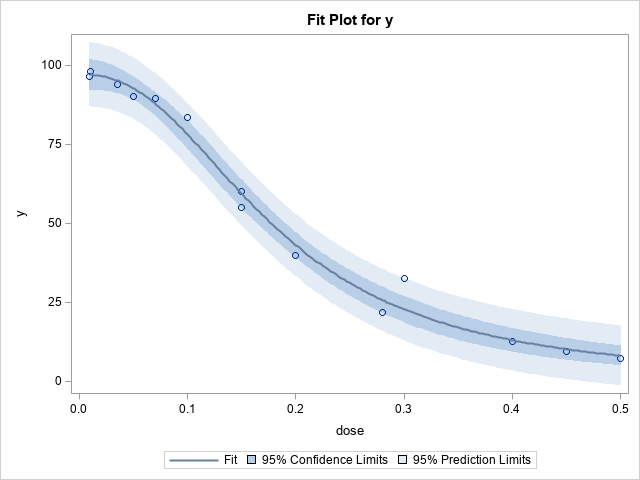The documentation for PROC NLIN contains an example of fitting a parametric dose-response curve to data. The example only has seven observations, but I have created an additional seven (fake) observations to make the example richer. The graph to the right visualizes the data and a parametric dose-response curve that is fit by using PROC NLIN. The curve represents the expected value of the log-logistic model
$f(x) = \delta + \frac{\alpha - \delta }{1+\gamma \exp \left\{ \beta \ln (x)\right\} }$
where x is the dose. The response is assumed to be bounded in the interval [δ, α], where δ is the response for x=0, and α is the limit of the response as x → ∞. The parameters β and γ determine the shape of the dose-response curve. In the following example, δ=0, and the data suggest that α ≈ 100.

The graph to the right demonstrates the data and the dose-response curve that best fits this data in a least-squares sense.

The following DATA step defines the data. The call to PROC NLIN specifies the model and produces the graph as well as a table of parameter estimates and the correlation between the regression parameters:

data DoseResponse; input dose y @@; logdose = log(dose); datalines; 0.009 96.56 0.035 94.12 0.07 89.76 0.15 60.21 0.20 39.95 0.28 21.88 0.50 7.46 0.01 98.1 0.05 90.2 0.10 83.6 0.15 55.1 0.30 32.5 0.40 12.8 0.45 9.6 ;   proc nlin data=DoseResponse plots(stats=none)=(fitplot); /* request the fit plot */ parameters alpha=100 beta=3 gamma=300; /* three parameter model */ delta = 0; /* a constant; not a parameter */ Switch = 1/(1+gamma*exp(beta*log(dose))); /* log-logistic function */ model y = delta + (alpha - delta)*Switch; run;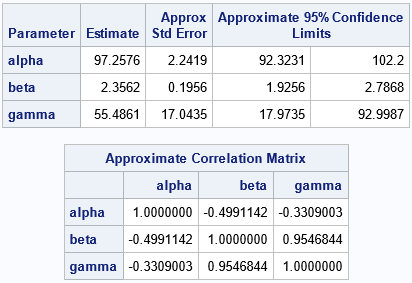Look at the statistics for the standard errors, the confidence intervals (CIs), and the correlation of the parameters. Notice that these columns contain the word "Approximate" (or "Approx") in the column headers. That is because these statistics are computed by using the formulas from linear regression models. Under the assumptions of ordinary linear regression, the estimates for the regression coefficients are (asymptotically) distributed according to a multivariate normal distribution. For nonlinear regression models, you do not know the sampling distribution of the parameter estimates in advance. However, you can use bootstrap methods to explore the sampling distribution and to improve the estimates of standard error, confidence intervals, and the correlation of the parameters.

### The BOOTSTRAP statement in PROC NLIN

You could carry out the bootstrap manually, but PROC NLIN supports the BOOTSTRAP statement, which automatically runs a bootstrap analysis for the regression coefficients. The BOOTSTRAP statement supports the following options:

• Use the SEED= option to specify the random-number stream.
• Use the NSAMPLES= option to specify the number of bootstrap samples that you want to use to make inferences. I suggest at least 1000, and 5000 or 10000 are better values.
• Use the BOOTCI option to request bootstrap confidence intervals for the regression parameters. You can use the BOOTCI(BC) suboption to request the bias-corrected and adjusted method. Use the BOOTCI(PERC) option for the traditional percentile-based confidence intervals. I recommend the bias-corrected option, which is the default.
• Use the BOOTCOV option to display a table that shows the estimated covariance between parameters.
• Use the BOOTPLOTS option to produce histograms of the bootstrap statistics for each regression parameter.

The following call to PROC NLIN repeats the previous analysis, but this time requests a bootstrap analysis to improve the estimates of standard error, CIs, and covariance between parameters:

proc nlin data=DoseResponse plots(stats=none)=(fitplot); /* request the fit plot */ parameters alpha=100 beta=3 gamma=300; /* three parameter model */ delta = 0; /* a constant; not a parameter */ Switch = 1/(1+gamma*exp(beta*log(dose))); /* log-logistic function */ model y = delta + (alpha - delta)*Switch; BOOTSTRAP / seed=12345 nsamples=5000 bootci(/*BC*/) bootcov bootplots; run; run;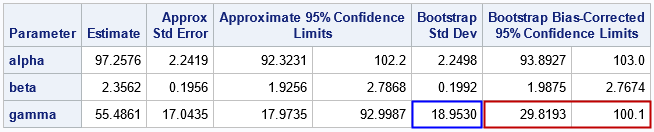The Parameter Estimates table is the most important output because it shows the bootstrap estimates for the standard error and CIs. For these data, you can see that the estimates for the alpha and beta parameters are not very different from the approximate estimates. However, the estimates for the gamma parameter are quite different. The bootstrap standard error is almost 2 units wider. The bootstrap bias-corrected confidence interval is about 5 units shorter and is shifted to the right as compared to the traditional CI.

You can study the bootstrap distribution of the gamma parameter to understand the differences. The following histogram is produced automatically by PROC NLIN because we used the BOOTPLOTS option. You can see that the distribution of the gamma estimates is not normal. It shows a moderate amount of positive skewness and kurtosis. Thus, the bootstrap estimates are noticeably different from the traditional estimates.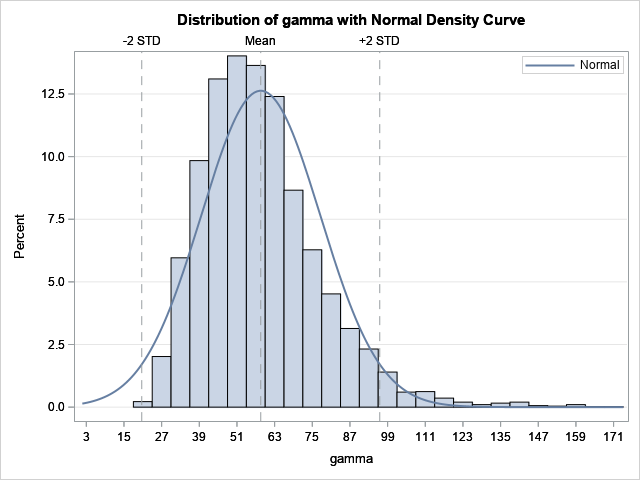The distributions for the alpha and beta statistics are not shown, but they display only small deviations from normality. Thus, the bootstrap estimates for the alpha and beta parameters are not very different from the traditional estimates.

I do not show the bootstrap estimates of the covariance of the parameters. However, if you convert the bootstrap estimates of covariance to a correlation matrix, you will find that the bootstrap estimates are close to the approximate estimates that are shown earlier.

### Summary

The BOOTSTRAP statement in PROC NLIN makes it easy to perform a bootstrap analysis of the regression estimates for a nonlinear regression model. The BOOTSTRAP statement will automatically produce bootstrap estimates of the standard error, confidence intervals, and covariance of parameters. In addition, you can use the BOOTPLOTS option to visualize the bootstrap distribution of the estimates. As shown in this example, sometimes the distribution of a parameter estimate is non-normal, so a bootstrap analysis produces better inferential statistics for the regression analysis.

The post Bootstrap estimates for nonlinear regression models in SAS appeared first on The DO Loop.

This article implements Passing-Bablok regression in SAS. Passing-Bablok regression is a one-variable regression technique that is used to compare measurements from different instruments or medical devices. The measurements of the two variables (X and Y) are both measured with errors. Consequently, you cannot use ordinary linear regression, which assumes that one variable (X) is measured without error. Passing-Bablok regression is a robust nonparametric regression method that does not make assumptions about the distribution of the expected values or the error terms in the model. It also does not assume that the errors are homoskedastic.

Several readers have asked about Passing-Bablok regression because I wrote an article about Deming regression, which is similar. On the SAS Support Communities, there was a lively discussion in response to a program by KSharp in which he shows how to use Base SAS procedures to perform a Passing-Bablok regression. In response to this interest, this article shares a SAS/IML program that performs Passing-Bablok regression.

### An overview of Passing-Bablok regression

Passing-Bablok regression (Passing and Bablok, 1983, J. Clin. Chem. Clin. Biochem., henceforth denote PB) fits a line through X and Y measurements. The X and Y variables measure the same quantity in the same units. Usually, X is the "gold standard" technique, whereas Y is a new method that is cheaper, more accurate, less invasive, or has some other favorable attributes. Whereas Deming regression assumes normal errors, Passing-Bablok regression can handle any error distribution provided that the measurement errors for both methods follow the same distribution. Also, the variance of each method does not need to be constant across the range of data. All that is required is that the ratio of the variances is constant. The Passing-Bablok method is robust to outliers in X or Y.

Assume you have N pairs of observations {(xi, yi), i=1..N}, where X and Y are different ways to measure the same quantity. Passing-Bablok regression determines whether the measurements methods are equivalent by estimating a line y = a + b*x. The estimate of the slope (b) is based on the median of pairs of slopes Sij = (yi - yj) / (xi - xj) for i < j. You must be careful when xi=xj. PB suggest assigning a large positive value when xi=xj and yi > yj and a large negative value when xi=xj and yi < yj. If xi=xj and yi=yj, the difference is not computed or used.

The Passing-Bablok method estimates the regression line y = a + b*x by using the following steps:
1. Compute all the pairwise slopes. For vertical slopes, use large positive or negative values, as appropriate.
2. Exclude slopes that are exactly -1. The reason is technical, but it involves ensuring the same conclusions whether you regress Y onto X or X onto Y.
3. Ideally, we would estimate the slope of the regression line (b) as the median of all the pairwise slopes of the points (xi, yi) and (xj, yj), where i < j. This is a robust statistic and is similar to Theil-Sen regression. However, the slopes are not independent, so the median can be a biased estimator. Instead, estimate the slope as the shifted median of the pairwise slopes.
4. Estimate the intercept, a, as the median of the values {yibxi}.
5. Use a combination of symmetry, rank-order statistics, and asymptotics to obtain confidence intervals (CIs) for b and a.
6. You can use the confidence intervals (CIs) to assess whether the two methods are equivalent. In terms of the regression estimates, the CI for the intercept (a) must contain 0 and the CI for the slope (b) must contain 1. This article uses only the CIs proposed by Passing and Bablok. Some software support jackknife or bootstrap CIs, but it is sometimes a bad idea to use resampling methods to estimate the CI of a median, so I do not recommend bootstrap CIs for Passing-Bablok regression.

### Implement Passing-Bablok regression in SAS

Because the Passing-Bablok method relies on computing vectors and medians, the SAS/IML language is a natural language for implementing the algorithm. The implementation uses several helper functions from previous articles:

The following modules implement the Passing-Bablok algorithm in SAS and store the modules for future use:

%let INFTY = 1E12; /* define a big number to use as "infinity" for vertical slopes */ proc iml; /* Extract only the values X[i,j] where i < j. https://blogs.sas.com/content/iml/2012/08/16/extract-the-lower-triangular-elements-of-a-matrix.html */ start StrictLowerTriangular(X); v = cusum( 1 || (ncol(X):2) ); return( remove(vech(X), v) ); finish; /* Compute pairwise differences: https://blogs.sas.com/content/iml/2011/02/09/computing-pairwise-differences-efficiently-of-course.html */ start PairwiseDiff(x); /* x is a column vector */ n = nrow(x); m = shape(x, n, n); /* duplicate data */ diff = m - m; /* pairwise differences */ return( StrictLowerTriangular(diff) ); finish;   /* Implement the Passing-Bablok (1983) regression method */ start PassingBablok(_x, _y, alpha=0.05, debug=0); keepIdx = loc(_x^=. & _y^=.); /* 1. remove missing values */ x = _x[keepIdx]; y = _y[keepIdx]; nObs = nrow(x); /* sample size of nonmissing values */   /* 1. Compute all the pairwise slopes. For vertical, use large positive or negative values. */ DX = T(PairwiseDiff(x)); /* x[i] - x[j] */ DY = T(PairwiseDiff(y)); /* y[i] - y[j] */   /* "Identical pairs of measurements with x_i=x_j and y_i=y_j do not contribute to the estimation of beta." (PB, p 711) */ idx = loc(DX^=0 | DY^=0); /* exclude 0/0 slopes (DX=0 and DY=0) */ G = DX[idx] || DY[idx] || j(ncol(idx), 1, .); /* last col is DY/DX */ idx = loc( G[,1] ^= 0 ); /* find where DX ^= 0 */ G[idx,3] = G[idx,2] / G[idx,1]; /* form Sij = DY / DX for DX^=0 */   /* Special cases: 1) x_i=x_j, but y_i^=y_j ==> Sij = +/- infinity 2) x_i^=x_j, but y_i=y_j ==> Sij = 0 "The occurrence of any of these special cases has a probability of zero (experimental data should exhibit these cases very rarely)."   Passing and Bablok do not specify how to handle, but one way is to keep these values within the set of valid slopes. Use large positive or negative numbers for +infty and -infty, respectively. */ posIdx = loc(G[,1]=0 & G[,2]>0); /* DX=0 & DY>0 */ if ncol(posIdx) then G[posIdx,3] = &INFTY; negIdx = loc(G[,1]=0 & G[,2]<0); /* DX=0 & DY<0 */ if ncol(negIdx) then G[negIdx,3] = -&INFTY; if debug then PRINT (T(1:nrow(G)) || G)[c={"N" "DX" "DY" "S"}];   /* 2. Exclude slopes that are exactly -1 */ /* We want to be able to interchange the X and Y variables. "For reasons of symmetry, any Sij with a value of -1 is also discarded." */ idx = loc(G[,3] ^= -1); S = G[idx,3]; N = nrow(S); /* number of valid slopes */ call sort(S); /* sort slopes ==> pctl computations easier */ if debug then print (S)[L='sort(S)'];   /* 3. Estimate the slope as the shifted median of the pairwise slopes. */ K = sum(S < -1); /* shift median by K values */ b = choose(mod(N,2), /* estimate of slope */ S[(N+1)/2+K ], /* if N odd, shifted median */ mean(S[N/2+K+{0,1}])); /* if N even, average of adjacent values */ /* 4. Estimate the intercept as the median of the values {yi – bxi}. */ a = median(y - b*x); /* estimate of the intercept, a */   /* 5. Estimate confidence intervals. */ C = quantile("Normal", 1-alpha/2) * sqrt(nObs*(nObs-1)*(2*nObs+5)/18); M1 = round((N - C)/2); M2 = N - M1 + 1; if debug then PRINT K C M1 M2 (M1+K)[L="M1+K"] (M2+k)[L="M1+K"]; CI_b = {. .}; /* initialize CI to missing */ if 1 <= M1+K & M1+K <= N then CI_b = S[M1+K]; if 1 <= M2+K & M2+K <= N then CI_b = S[M2+K]; CI_a = median(y - CI_b[,2:1]@x); /* aL=med(y-bU*x); aU=med(y-bL*x); */   /* return a list that contains the results */ outList = [#'a'=a, #'b'=b, #'CI_a'=CI_a, #'CI_b'=CI_b]; /* pack into list */ return( outList ); finish;   store module=(StrictLowerTriangular PairwiseDiff PassingBablok); quit;

Notice that the PassingBablok function returns a list. A list is a convenient way to return multiple statistics from a function.

### Perform Passing-Bablok regression in SAS

Having saved the SAS/IML modules, let's see how to call the PassingBablok routine and analyze the results. The following DATA step defines 102 pairs of observations. These data were posted to the SAS Support Communities by @soulmate. To support running regressions on multiple data sets, I use macro variables to store the name of the data set and the variable names.

data PBData; label x="Method 1" y="Method 2"; input x y @@; datalines; 0.07 0.07 0.04 0.10 0.07 0.08 0.05 0.10 0.06 0.08 0.06 0.07 0.03 0.05 0.04 0.05 0.05 0.08 0.05 0.10 0.12 0.21 0.10 0.17 0.03 0.11 0.18 0.24 0.29 0.33 0.05 0.05 0.04 0.05 0.11 0.21 0.38 0.36 0.04 0.05 0.03 0.06 0.06 0.07 0.46 0.27 0.08 0.05 0.02 0.09 0.12 0.23 0.16 0.25 0.07 0.10 0.07 0.07 0.04 0.06 0.11 0.23 0.23 0.21 0.15 0.20 0.05 0.07 0.04 0.14 0.05 0.11 0.17 0.26 8.43 8.12 1.86 1.47 0.17 0.27 8.29 7.71 5.98 5.26 0.57 0.43 0.07 0.10 0.06 0.11 0.21 0.24 8.29 7.84 0.28 0.23 8.25 7.79 0.18 0.25 0.17 0.22 0.15 0.21 8.33 7.67 5.58 5.05 0.09 0.14 1.00 3.72 0.09 0.13 3.88 3.54 0.51 0.42 4.09 3.64 0.89 0.71 5.61 5.18 4.52 4.15 0.09 0.12 0.05 0.05 0.05 0.05 0.04 0.07 0.05 0.07 0.09 0.11 0.03 0.05 2.27 2.08 1.50 1.21 5.05 4.46 0.22 0.25 2.13 1.93 0.05 0.08 4.09 3.61 1.46 1.13 1.20 0.97 0.02 0.05 1.00 1.00 3.39 3.11 1.00 0.05 2.07 1.83 6.68 6.06 3.00 2.97 0.06 0.09 7.17 6.55 1.00 1.00 2.00 0.05 2.91 2.45 3.92 3.36 1.00 1.00 7.20 6.88 6.42 5.83 2.38 2.04 1.97 1.76 4.72 4.37 1.64 1.25 5.48 4.77 5.54 5.16 0.02 0.06 ;   %let DSName = PBData; /* name of data set */ %let XVar = x; /* name of X variable (Method 1) */ %let YVar = y; /* name of Y variable (Method 2) */   title "Measurements from Two Methods"; proc sgplot data=&DSName; scatter x=&XVar y=&YVar; lineparm x=0 y=0 slope=1 / clip; /* identity line: Intercept=0, Slope=1 */ xaxis grid; yaxis grid; run;The graph indicates that the two measurements are linearly related and strongly correlated. It looks like Method 1 (X) produces higher measurements than Method 2 (Y) because most points are to the right of the identity line. Three outliers are visible. The Passing-Bablok regression should be robust to these outliers.

The following SAS/IML program analyzes the data by calling the PassingBablok function:

proc iml; load module=(StrictLowerTriangular PairwiseDiff PassingBablok); use &DSName; read all var {"&XVar"} into x; read all var {"&YVar"} into y; close;   R = PassingBablok(x, y); ParameterEstimates = (R$'a' || R$'CI_a') // /* create table from the list items */ (R$'b' || R$'CI_b'); print ParameterEstimates[c={'Estimate' 'LCL' 'UCL'} r={'Intercept' 'Slope'}];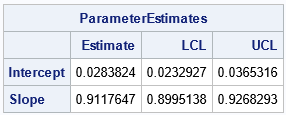The program reads the X and Y variables and calls the PassingBablok function, which returns a list of statistics. You can use the ListPrint subroutine to print the items in the list, or you can extract the items and put them into a matrix, as in the example.

The output shows that the Passing-Bablok regression line is Y = 0.028 + 0.912*X. The confidence interval for the slope parameter (b) does NOT include 1, which means that we reject the null hypothesis that the measurements are related by a line that has unit slope. Even if the CI for the slope had contained 1, the CI for the Intercept (a) does not include 0. For these data, we reject the hypothesis that the measurements from the two methods are equivalent.

Of course, you could encapsulate the program into a SAS macro that performs Passing-Bablok regression.

### A second example of Passing-Bablok regression in SAS

For completeness, the following example shows results for two methods that are equivalent. The output shows that the CI for the slope includes 1 and the CI for the intercept includes 0:

%let DSName = PBData2; /* name of data set */ %let XVar = m1; /* name of X variable (Method 1) */ %let YVar = m2; /* name of Y variable (Method 2) */   data &DSName; label x="Method 1" y="Method 2"; input m1 m2 @@; datalines; 10.1 10.1 10.5 10.6 12.8 13.1 8.7 8.7 10.8 11.5 10.6 10.4 9.6 9.9 11.3 11.3 7.7 7.5 11.5 11.9 7.9 7.8 9.9 9.7 9.3 9.9 16.3 16.2 9.4 9.8 11.0 11.8 9.1 8.7 12.2 11.9 13.4 12.6 9.2 9.5 8.8 9.1 9.3 9.2 8.5 8.7 9.6 9.7 13.5 13.1 9.4 9.1 9.5 9.6 10.8 11.3 14.6 14.8 9.7 9.3 16.4 16.5 8.1 8.6 8.3 8.1 9.5 9.6 20.3 20.4 8.6 8.5 9.1 8.8 8.8 8.7 9.2 9.9 8.1 8.1 9.2 9.0 17.0 17.0 10.2 10.0 10.0 9.8 6.5 6.6 7.9 7.6 11.3 10.7 14.2 14.1 11.9 12.7 9.9 9.4 ;   proc iml; load module=(StrictLowerTriangular PairwiseDiff PassingBablok); use &DSName; read all var {"&XVar"} into x; read all var {"&YVar"} into y; close;   R = PassingBablok(x, y); ParameterEstimates = (R$'a' || R$'CI_a') // (R$'b' || R$'CI_b'); print ParameterEstimates[c={'Estimate' 'LCL' 'UCL'} r={'Intercept' 'Slope'}]; QUIT;`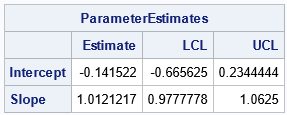For these data, the Passing-Bablok regression line is Y = 0.028 + 0.912*X. The confidence interval for the Slope term is [0.98, 1.06], which contains 1. The confidence interval for the Intercept is [-0.67, 0.23], which contains 0. Thus, these data suggest that the two methods are equivalent ways to measure the same quantity.

### Summary

This article shows how to implement Passing-Bablok regression in SAS by using the SAS/IML language. It also explains how Passing-Bablok regression works and why different software might provide different confidence intervals. You can download the complete SAS program that implements Passing-Bablok regression and creates the graphs and tables in this article.